Скачать презентацию Introduction to Information Security Lecture 4 Public Key

0f19ecb88202de98e3fb1499c6c24afa.ppt

• Количество слайдов: 72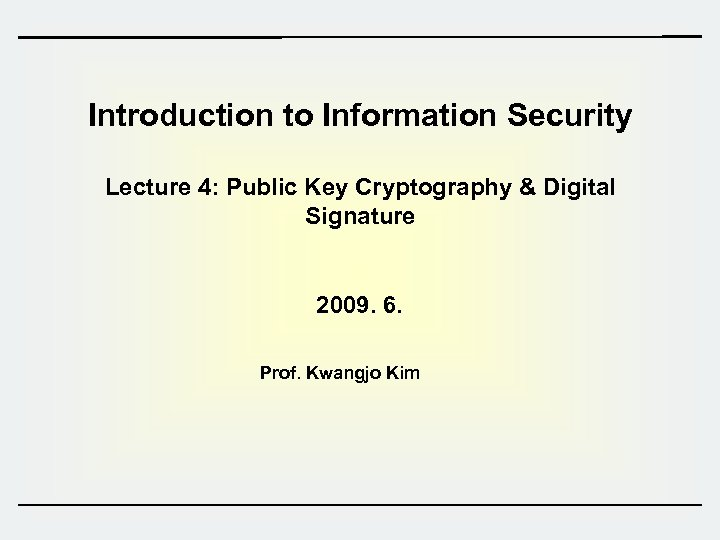Introduction to Information Security Lecture 4: Public Key Cryptography & Digital Signature 2009. 6. Prof. Kwangjo Kim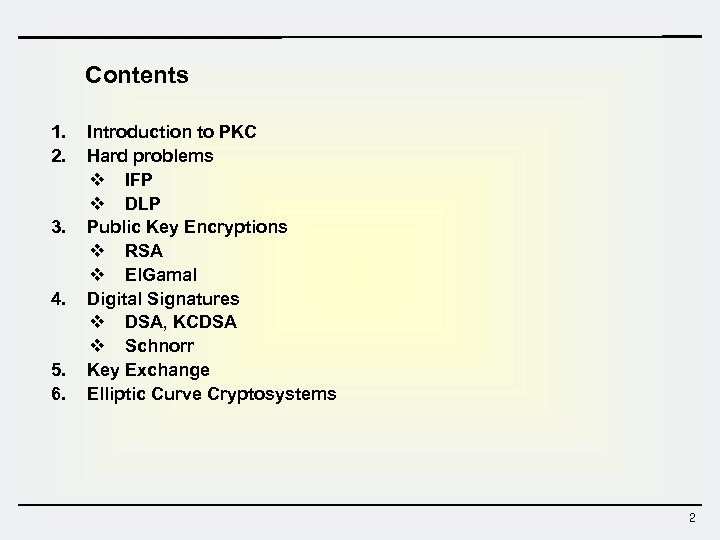Contents 1. 2. 3. 4. 5. 6. Introduction to PKC Hard problems v IFP v DLP Public Key Encryptions v RSA v El. Gamal Digital Signatures v DSA, KCDSA v Schnorr Key Exchange Elliptic Curve Cryptosystems 2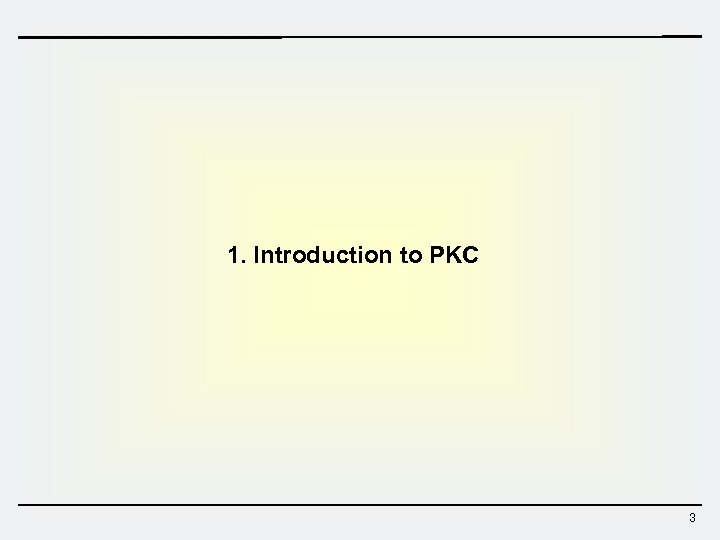1. Introduction to PKC 3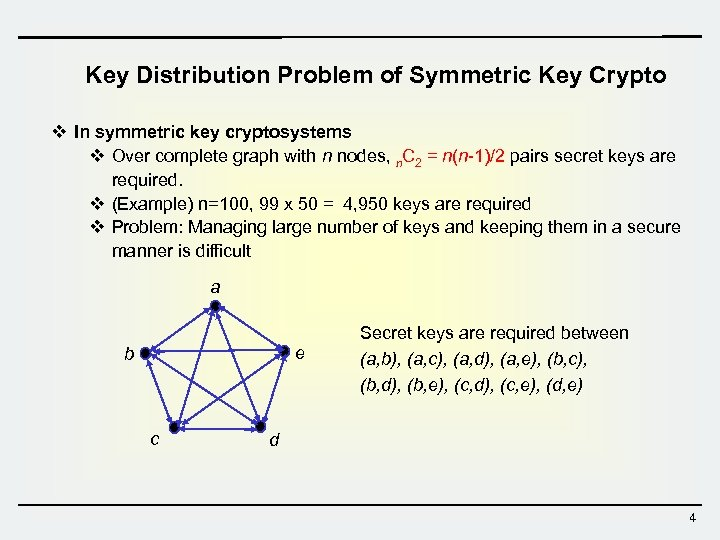Key Distribution Problem of Symmetric Key Crypto v In symmetric key cryptosystems v Over complete graph with n nodes, n. C 2 = n(n-1)/2 pairs secret keys are required. v (Example) n=100, 99 x 50 = 4, 950 keys are required v Problem: Managing large number of keys and keeping them in a secure manner is difficult a e b c Secret keys are required between (a, b), (a, c), (a, d), (a, e), (b, c), (b, d), (b, e), (c, d), (c, e), (d, e) d 4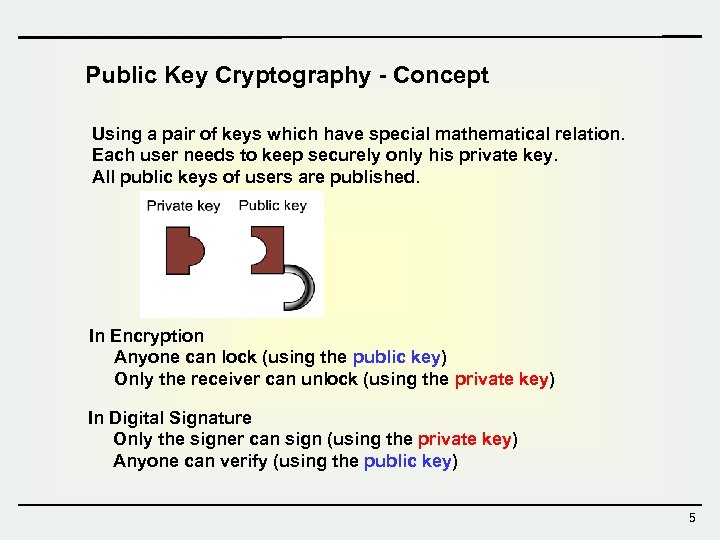Public Key Cryptography - Concept Using a pair of keys which have special mathematical relation. Each user needs to keep securely only his private key. All public keys of users are published. In Encryption Anyone can lock (using the public key) Only the receiver can unlock (using the private key) In Digital Signature Only the signer can sign (using the private key) Anyone can verify (using the public key) 5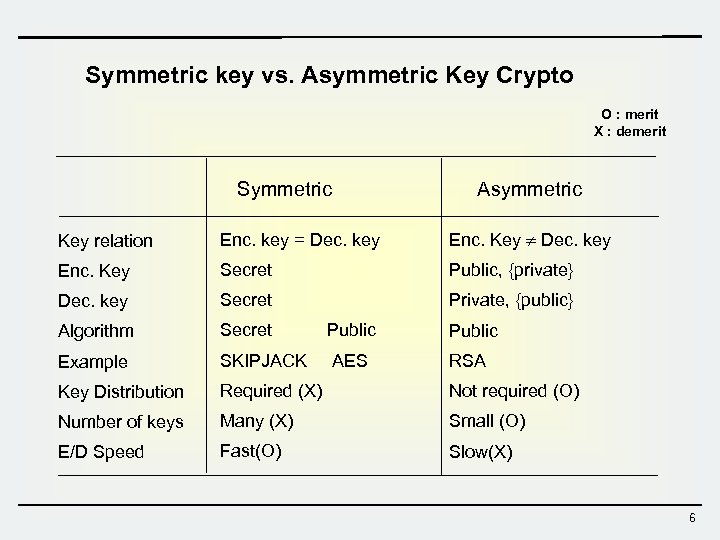Symmetric key vs. Asymmetric Key Crypto O : merit X : demerit Symmetric Asymmetric Key relation Enc. key = Dec. key Enc. Key Secret Public, {private} Dec. key Secret Private, {public} Algorithm Secret Public Example SKIPJACK AES RSA Key Distribution Required (X) Not required (O) Number of keys Many (X) Small (O) E/D Speed Fast(O) Slow(X) 6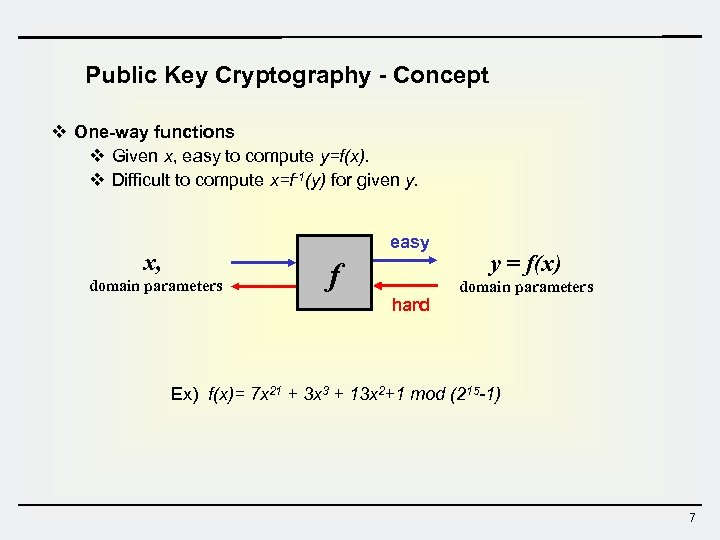Public Key Cryptography - Concept v One-way functions v Given x, easy to compute y=f(x). v Difficult to compute x=f-1(y) for given y. easy x, domain parameters f hard y = f(x) domain parameters Ex) f(x)= 7 x 21 + 3 x 3 + 13 x 2+1 mod (215 -1) 7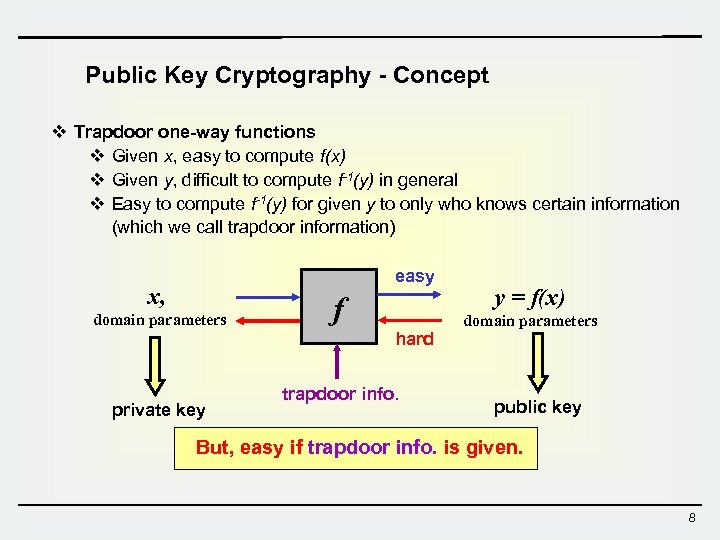Public Key Cryptography - Concept v Trapdoor one-way functions v Given x, easy to compute f(x) v Given y, difficult to compute f-1(y) in general v Easy to compute f-1(y) for given y to only who knows certain information (which we call trapdoor information) easy x, domain parameters f hard private key trapdoor info. y = f(x) domain parameters public key But, easy if trapdoor info. is given. 8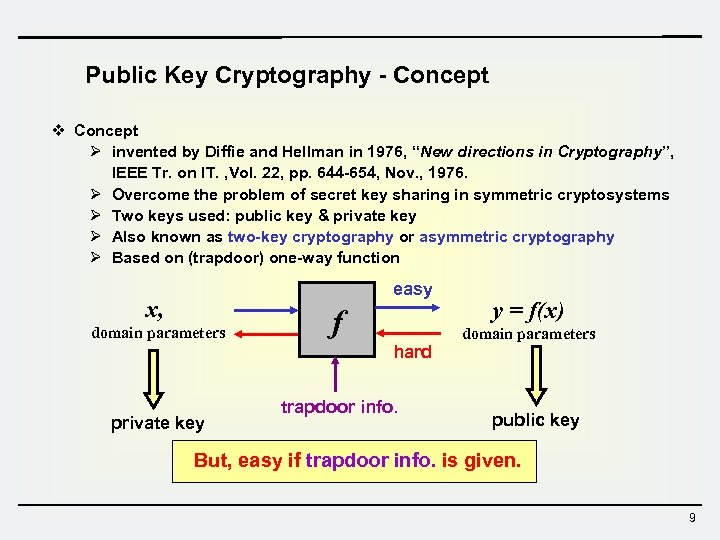Public Key Cryptography - Concept v Concept Ø invented by Diffie and Hellman in 1976, “New directions in Cryptography”, IEEE Tr. on IT. , Vol. 22, pp. 644 -654, Nov. , 1976. Ø Overcome the problem of secret key sharing in symmetric cryptosystems Ø Two keys used: public key & private key Ø Also known as two-key cryptography or asymmetric cryptography Ø Based on (trapdoor) one-way function easy x, domain parameters f hard private key trapdoor info. y = f(x) domain parameters public key But, easy if trapdoor info. is given. 9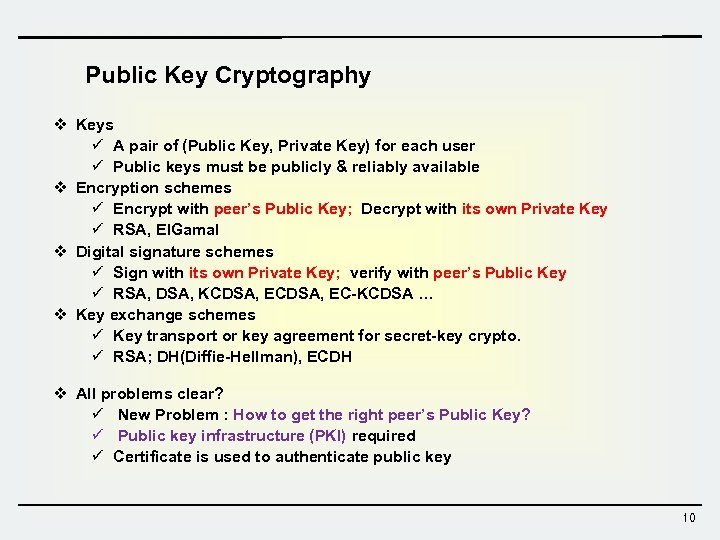Public Key Cryptography v Keys ü A pair of (Public Key, Private Key) for each user ü Public keys must be publicly & reliably available v Encryption schemes ü Encrypt with peer’s Public Key; Decrypt with its own Private Key ü RSA, El. Gamal v Digital signature schemes ü Sign with its own Private Key; verify with peer’s Public Key ü RSA, DSA, KCDSA, EC-KCDSA … v Key exchange schemes ü Key transport or key agreement for secret-key crypto. ü RSA; DH(Diffie-Hellman), ECDH v All problems clear? ü New Problem : How to get the right peer’s Public Key? ü Public key infrastructure (PKI) required ü Certificate is used to authenticate public key 10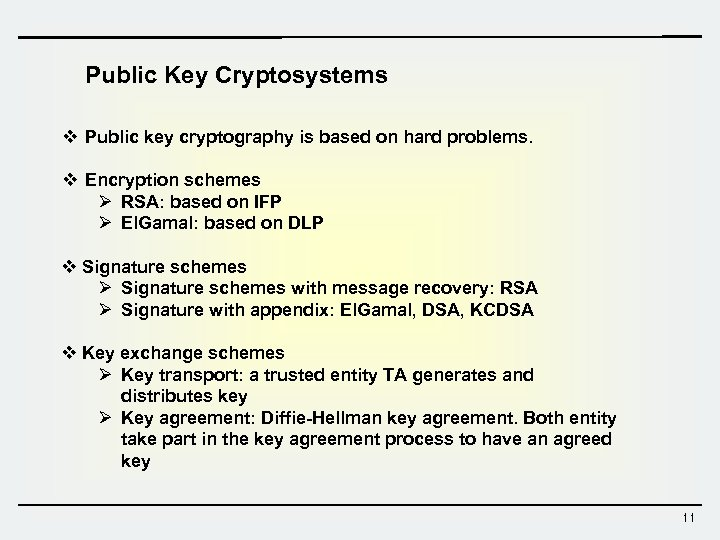Public Key Cryptosystems v Public key cryptography is based on hard problems. v Encryption schemes Ø RSA: based on IFP Ø El. Gamal: based on DLP v Signature schemes Ø Signature schemes with message recovery: RSA Ø Signature with appendix: El. Gamal, DSA, KCDSA v Key exchange schemes Ø Key transport: a trusted entity TA generates and distributes key Ø Key agreement: Diffie-Hellman key agreement. Both entity take part in the key agreement process to have an agreed key 11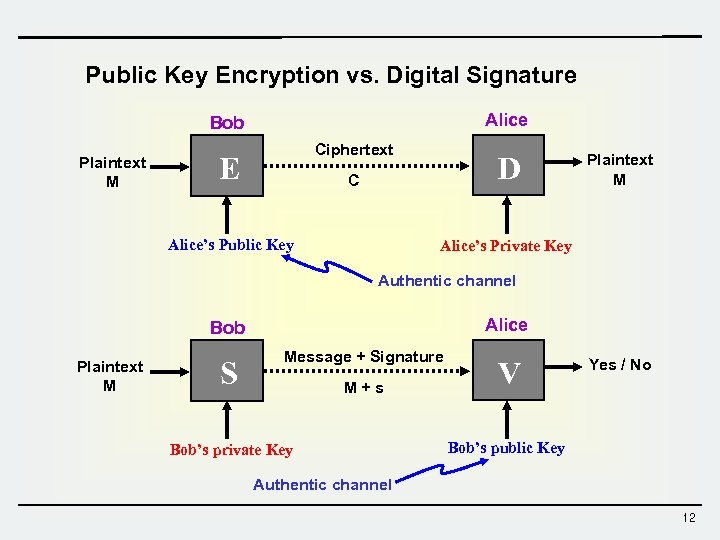Public Key Encryption vs. Digital Signature Alice Bob Plaintext M Ciphertext E D C Alice’s Public Key Plaintext M Alice’s Private Key Authentic channel Alice Bob Plaintext M S Message + Signature M+s Bob’s private Key V Yes / No Bob’s public Key Authentic channel 12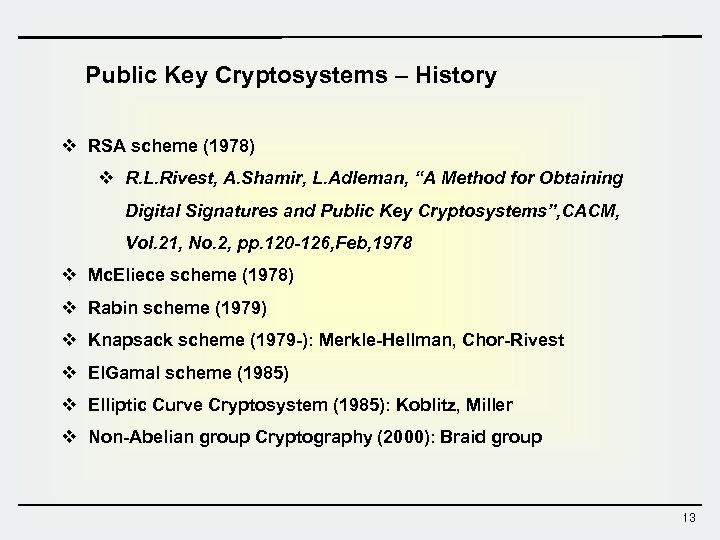Public Key Cryptosystems – History v RSA scheme (1978) v R. L. Rivest, A. Shamir, L. Adleman, “A Method for Obtaining Digital Signatures and Public Key Cryptosystems”, CACM, Vol. 21, No. 2, pp. 120 -126, Feb, 1978 v Mc. Eliece scheme (1978) v Rabin scheme (1979) v Knapsack scheme (1979 -): Merkle-Hellman, Chor-Rivest v El. Gamal scheme (1985) v Elliptic Curve Cryptosystem (1985): Koblitz, Miller v Non-Abelian group Cryptography (2000): Braid group 13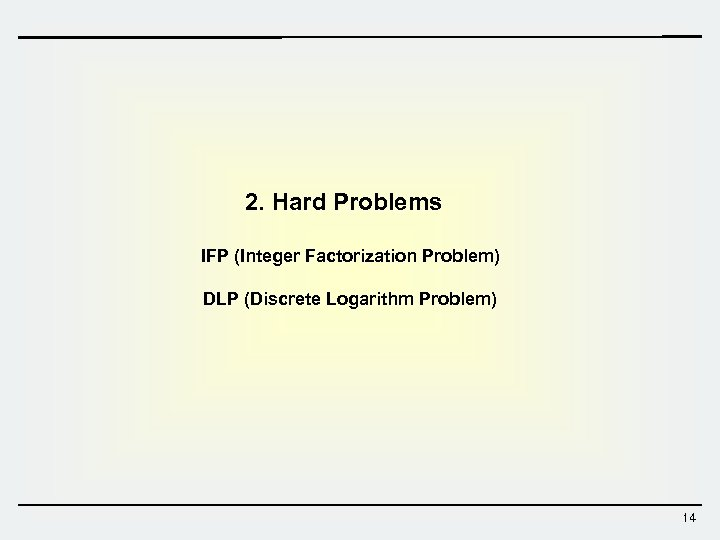2. Hard Problems IFP (Integer Factorization Problem) DLP (Discrete Logarithm Problem) 14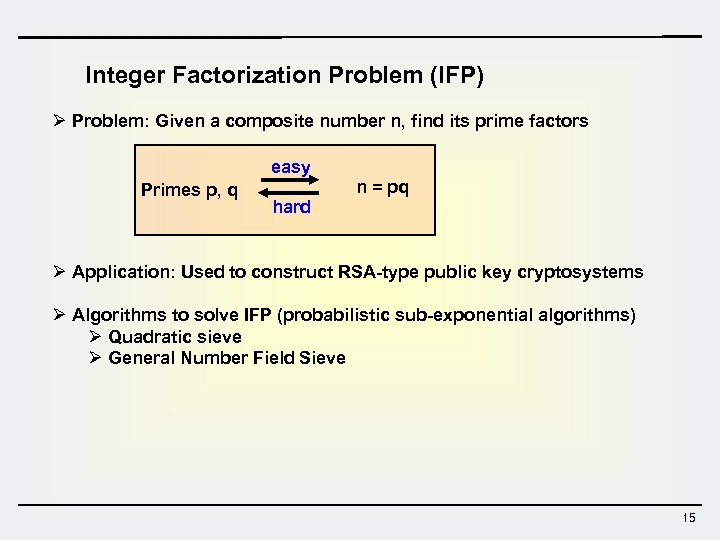Integer Factorization Problem (IFP) Ø Problem: Given a composite number n, find its prime factors easy Primes p, q n = pq hard Ø Application: Used to construct RSA-type public key cryptosystems Ø Algorithms to solve IFP (probabilistic sub-exponential algorithms) Ø Quadratic sieve Ø General Number Field Sieve 15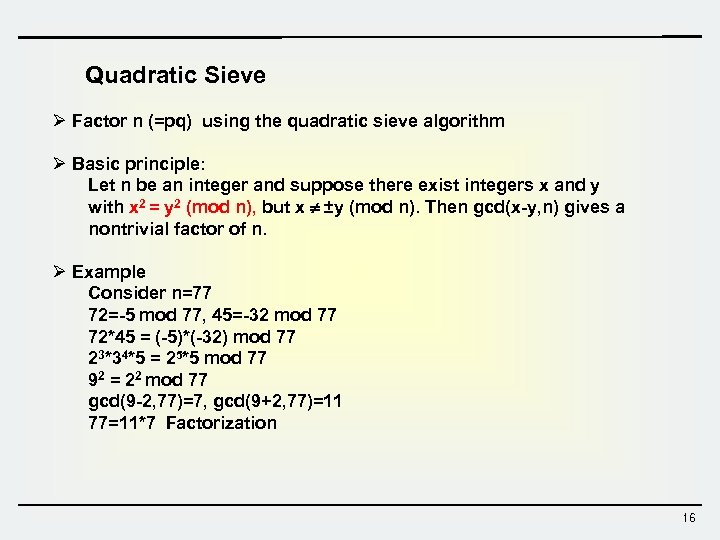Quadratic Sieve Ø Factor n (=pq) using the quadratic sieve algorithm Ø Basic principle: Let n be an integer and suppose there exist integers x and y with x 2 = y 2 (mod n), but x ±y (mod n). Then gcd(x-y, n) gives a nontrivial factor of n. Ø Example Consider n=77 72=-5 mod 77, 45=-32 mod 77 72*45 = (-5)*(-32) mod 77 23*34*5 = 25*5 mod 77 92 = 22 mod 77 gcd(9 -2, 77)=7, gcd(9+2, 77)=11 77=11*7 Factorization 16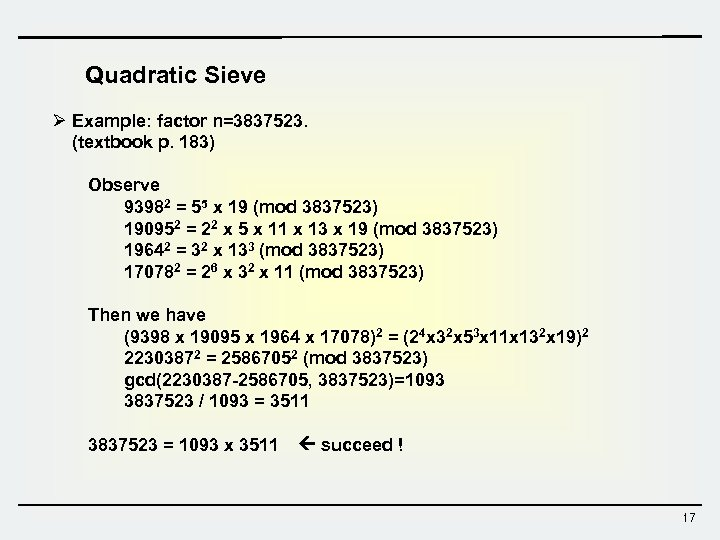Quadratic Sieve Ø Example: factor n=3837523. (textbook p. 183) Observe 93982 = 55 x 19 (mod 3837523) 190952 = 22 x 5 x 11 x 13 x 19 (mod 3837523) 19642 = 32 x 133 (mod 3837523) 170782 = 26 x 32 x 11 (mod 3837523) Then we have (9398 x 19095 x 1964 x 17078)2 = (24 x 32 x 53 x 11 x 132 x 19)2 22303872 = 25867052 (mod 3837523) gcd(2230387 -2586705, 3837523)=1093 3837523 / 1093 = 3511 3837523 = 1093 x 3511 succeed ! 17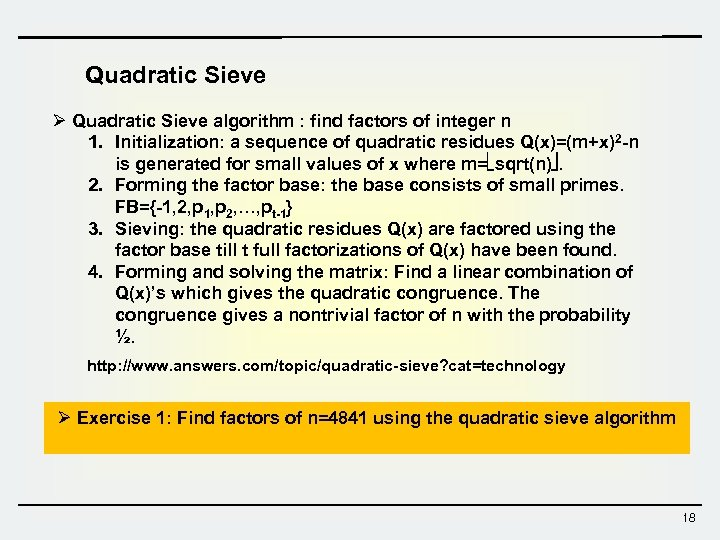Quadratic Sieve Ø Quadratic Sieve algorithm : find factors of integer n 1. Initialization: a sequence of quadratic residues Q(x)=(m+x)2 -n is generated for small values of x where m= sqrt(n). 2. Forming the factor base: the base consists of small primes. FB={-1, 2, p 1, p 2, …, pt-1} 3. Sieving: the quadratic residues Q(x) are factored using the factor base till t full factorizations of Q(x) have been found. 4. Forming and solving the matrix: Find a linear combination of Q(x)’s which gives the quadratic congruence. The congruence gives a nontrivial factor of n with the probability ½. http: //www. answers. com/topic/quadratic-sieve? cat=technology Ø Exercise 1: Find factors of n=4841 using the quadratic sieve algorithm 18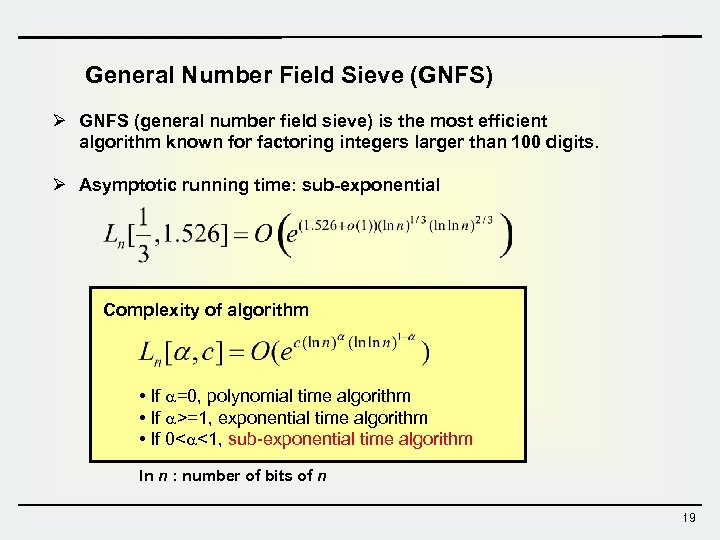General Number Field Sieve (GNFS) Ø GNFS (general number field sieve) is the most efficient algorithm known for factoring integers larger than 100 digits. Ø Asymptotic running time: sub-exponential Complexity of algorithm • If =0, polynomial time algorithm • If >=1, exponential time algorithm • If 0< <1, sub-exponential time algorithm ln n : number of bits of n 19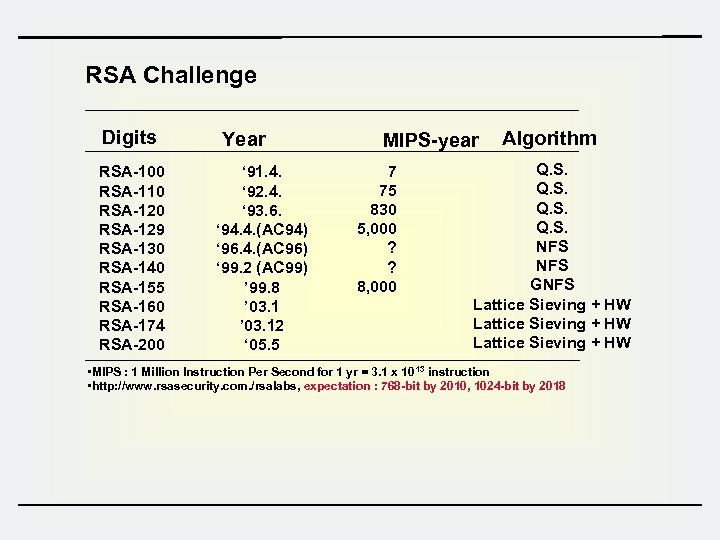RSA Challenge Digits RSA-100 RSA-110 RSA-129 RSA-130 RSA-140 RSA-155 RSA-160 RSA-174 RSA-200 Year ‘ 91. 4. ‘ 92. 4. ‘ 93. 6. ‘ 94. 4. (AC 94) ‘ 96. 4. (AC 96) ‘ 99. 2 (AC 99) ’ 99. 8 ’ 03. 12 ‘ 05. 5 MIPS-year 7 75 830 5, 000 ? ? 8, 000 Algorithm Q. S. NFS GNFS Lattice Sieving + HW • MIPS : 1 Million Instruction Per Second for 1 yr = 3. 1 x 10 13 instruction • http: //www. rsasecurity. com. /rsalabs, expectation : 768 -bit by 2010, 1024 -bit by 2018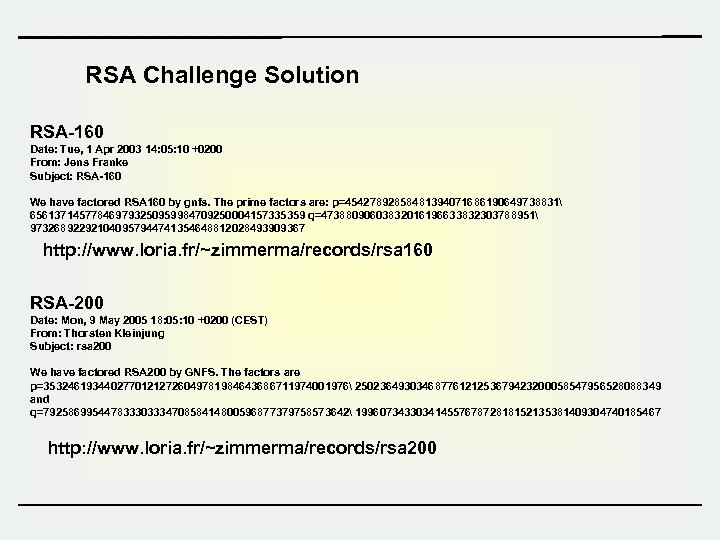RSA Challenge Solution RSA-160 Date: Tue, 1 Apr 2003 14: 05: 10 +0200 From: Jens Franke Subject: RSA-160 We have factored RSA 160 by gnfs. The prime factors are: p=45427892858481394071686190649738831 656137145778469793250959984709250004157335359 q=47388090603832016196633832303788951 973268922921040957944741354648812028493909367 http: //www. loria. fr/~zimmerma/records/rsa 160 RSA-200 Date: Mon, 9 May 2005 18: 05: 10 +0200 (CEST) From: Thorsten Kleinjung Subject: rsa 200 We have factored RSA 200 by GNFS. The factors are p=35324619344027701212726049781984643686711974001976 25023649303468776121253679423200058547956528088349 and q=79258699544783330333470858414800596877379758573642 19960734330341455767872818152135381409304740185467 http: //www. loria. fr/~zimmerma/records/rsa 200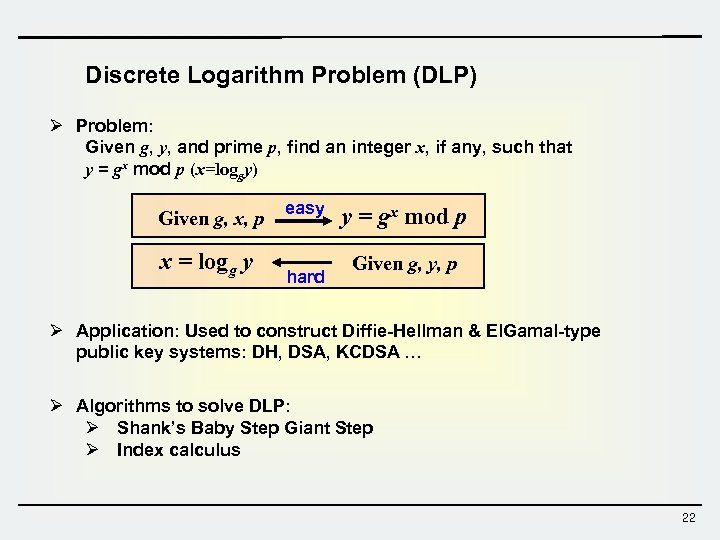Discrete Logarithm Problem (DLP) Ø Problem: Given g, y, and prime p, find an integer x, if any, such that y = gx mod p (x=loggy) Given g, x, p x = logg y easy hard y = gx mod p Given g, y, p Ø Application: Used to construct Diffie-Hellman & El. Gamal-type public key systems: DH, DSA, KCDSA … Ø Algorithms to solve DLP: Ø Shank’s Baby Step Giant Step Ø Index calculus 22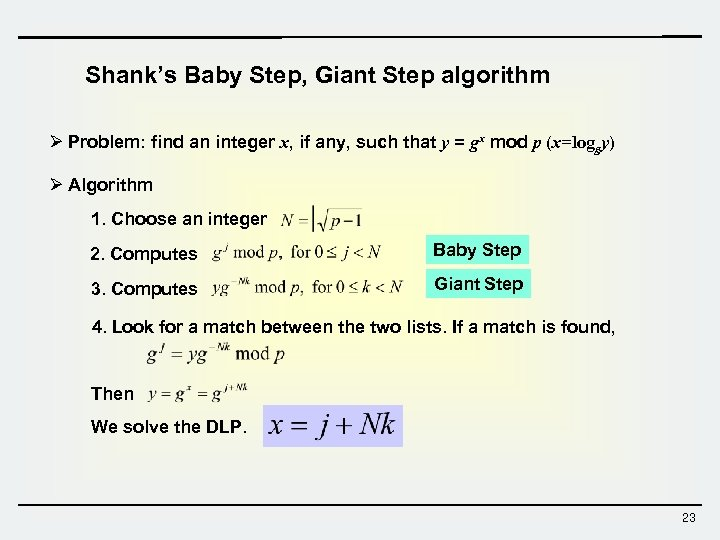Shank’s Baby Step, Giant Step algorithm Ø Problem: find an integer x, if any, such that y = gx mod p (x=loggy) Ø Algorithm 1. Choose an integer 2. Computes Baby Step 3. Computes Giant Step 4. Look for a match between the two lists. If a match is found, Then We solve the DLP. 23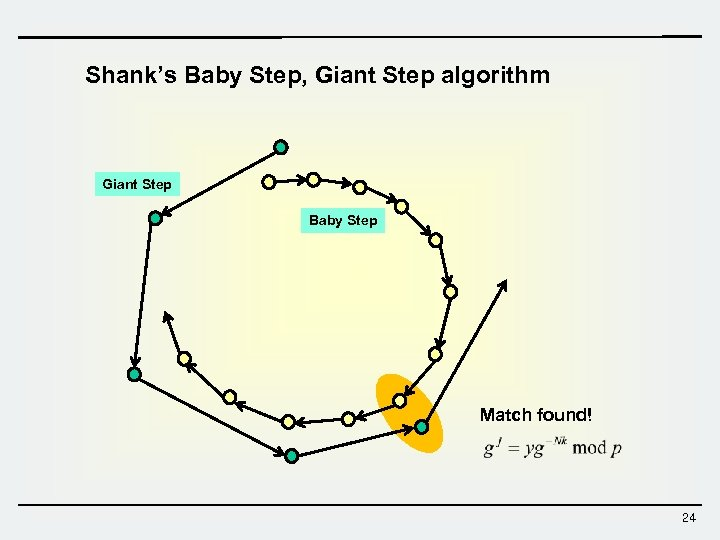Shank’s Baby Step, Giant Step algorithm Giant Step Baby Step Match found! 24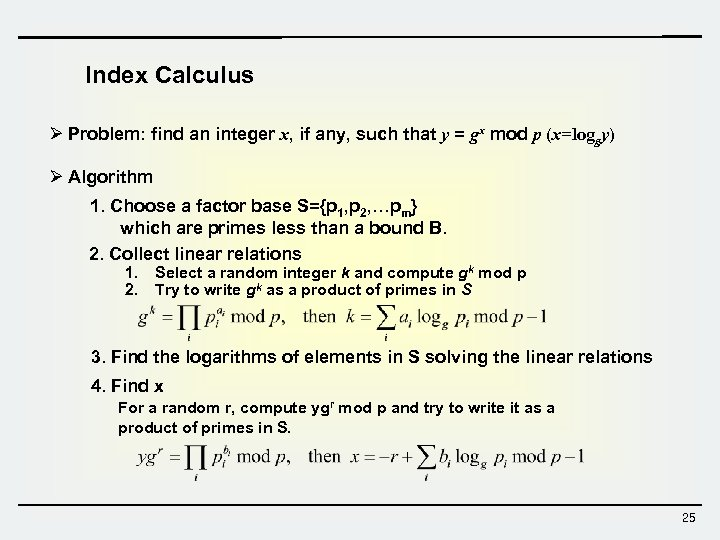Index Calculus Ø Problem: find an integer x, if any, such that y = gx mod p (x=loggy) Ø Algorithm 1. Choose a factor base S={p 1, p 2, …pm} which are primes less than a bound B. 2. Collect linear relations 1. Select a random integer k and compute gk mod p 2. Try to write gk as a product of primes in S 3. Find the logarithms of elements in S solving the linear relations 4. Find x For a random r, compute ygr mod p and try to write it as a product of primes in S. 25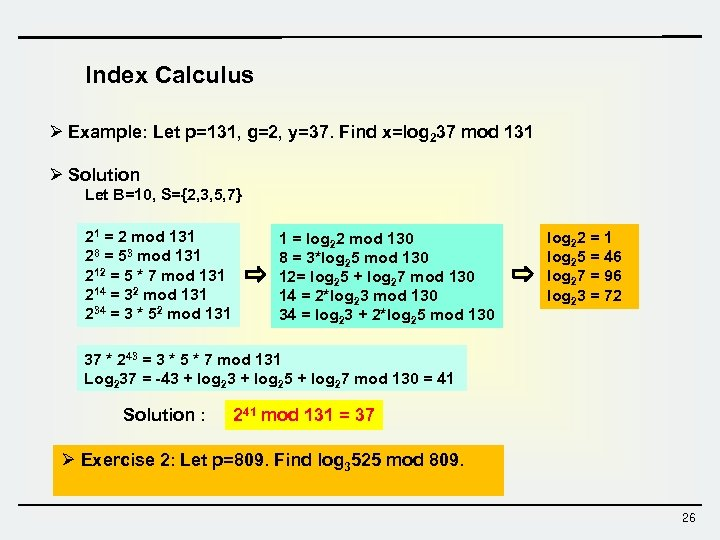Index Calculus Ø Example: Let p=131, g=2, y=37. Find x=log 237 mod 131 Ø Solution Let B=10, S={2, 3, 5, 7} 21 = 2 mod 131 28 = 53 mod 131 212 = 5 * 7 mod 131 214 = 32 mod 131 234 = 3 * 52 mod 131 1 = log 22 mod 130 8 = 3*log 25 mod 130 12= log 25 + log 27 mod 130 14 = 2*log 23 mod 130 34 = log 23 + 2*log 25 mod 130 log 22 = 1 log 25 = 46 log 27 = 96 log 23 = 72 37 * 243 = 3 * 5 * 7 mod 131 Log 237 = -43 + log 25 + log 27 mod 130 = 41 Solution : 241 mod 131 = 37 Ø Exercise 2: Let p=809. Find log 3525 mod 809. 26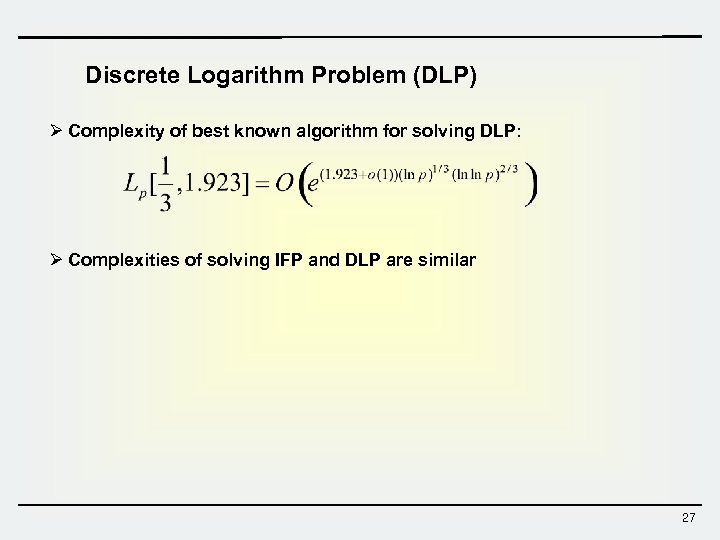Discrete Logarithm Problem (DLP) Ø Complexity of best known algorithm for solving DLP: Ø Complexities of solving IFP and DLP are similar 27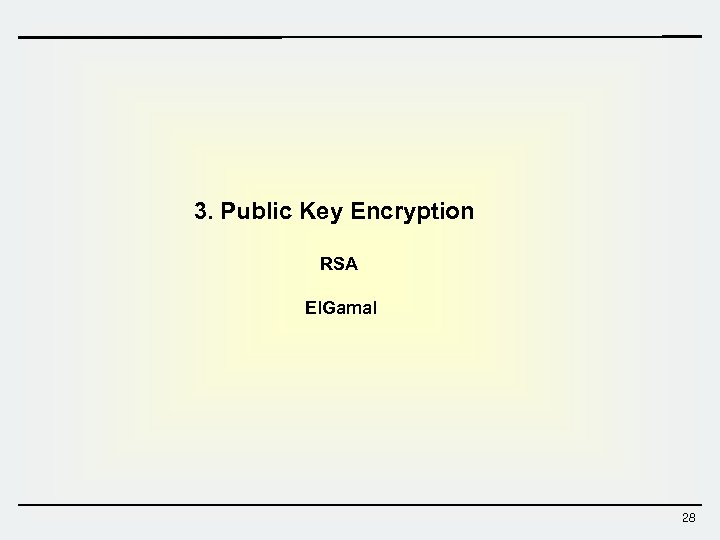3. Public Key Encryption RSA El. Gamal 28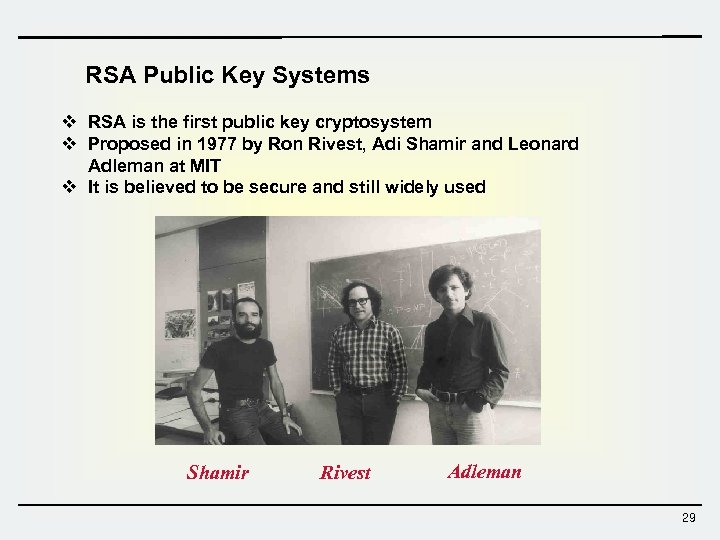RSA Public Key Systems v RSA is the first public key cryptosystem v Proposed in 1977 by Ron Rivest, Adi Shamir and Leonard Adleman at MIT v It is believed to be secure and still widely used Shamir Rivest Adleman 29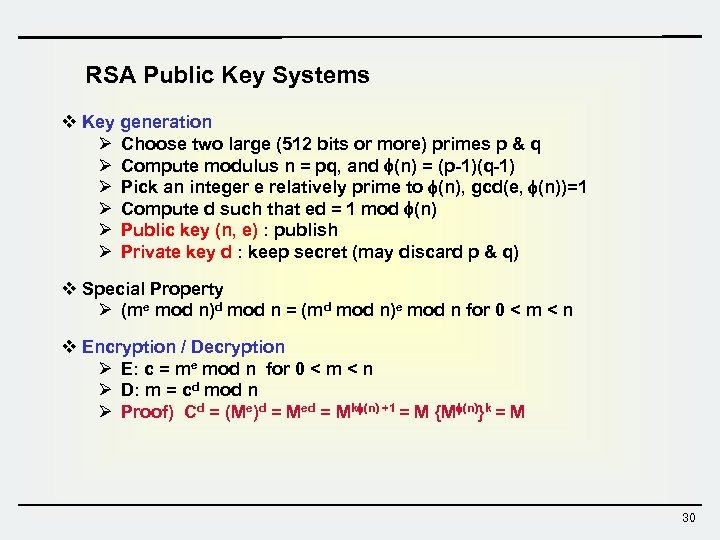RSA Public Key Systems v Key generation Ø Choose two large (512 bits or more) primes p & q Ø Compute modulus n = pq, and (n) = (p-1)(q-1) Ø Pick an integer e relatively prime to (n), gcd(e, (n))=1 Ø Compute d such that ed = 1 mod (n) Ø Public key (n, e) : publish Ø Private key d : keep secret (may discard p & q) v Special Property Ø (me mod n)d mod n = (md mod n)e mod n for 0 < m < n v Encryption / Decryption Ø E: c = me mod n for 0 < m < n Ø D: m = cd mod n Ø Proof) Cd = (Me)d = Med = Mk (n) +1 = M {M (n)}k = M 30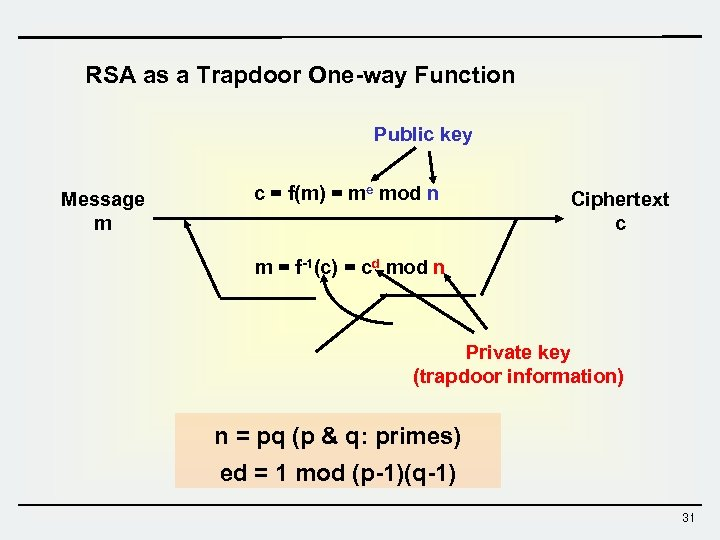RSA as a Trapdoor One-way Function Public key Message m c = f(m) = me mod n Ciphertext c m = f-1(c) = cd mod n Private key (trapdoor information) n = pq (p & q: primes) ed = 1 mod (p-1)(q-1) 31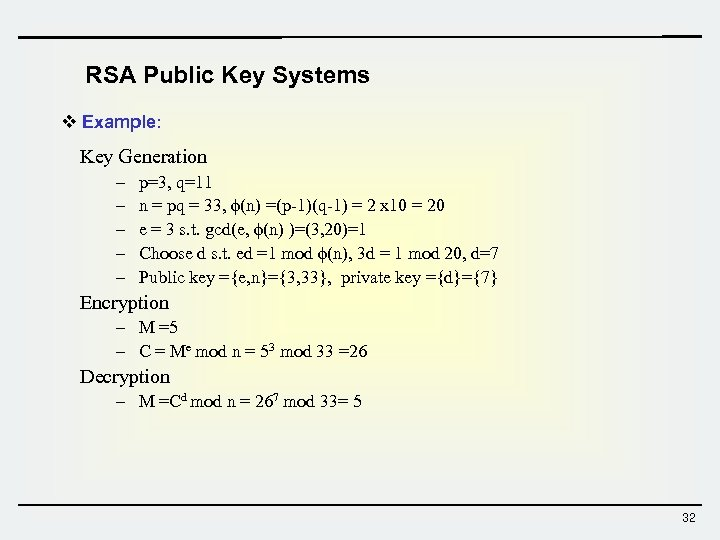RSA Public Key Systems v Example: Key Generation – – – p=3, q=11 n = pq = 33, (n) =(p-1)(q-1) = 2 x 10 = 20 e = 3 s. t. gcd(e, (n) )=(3, 20)=1 Choose d s. t. ed =1 mod (n), 3 d = 1 mod 20, d=7 Public key ={e, n}={3, 33}, private key ={d}={7} Encryption – M =5 – C = Me mod n = 53 mod 33 =26 Decryption – M =Cd mod n = 267 mod 33= 5 32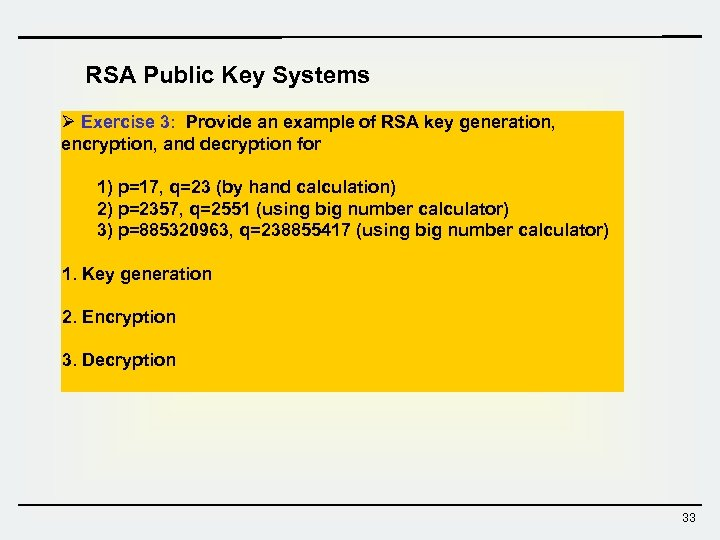RSA Public Key Systems Ø Exercise 3: Provide an example of RSA key generation, encryption, and decryption for 1) p=17, q=23 (by hand calculation) 2) p=2357, q=2551 (using big number calculator) 3) p=885320963, q=238855417 (using big number calculator) 1. Key generation 2. Encryption 3. Decryption 33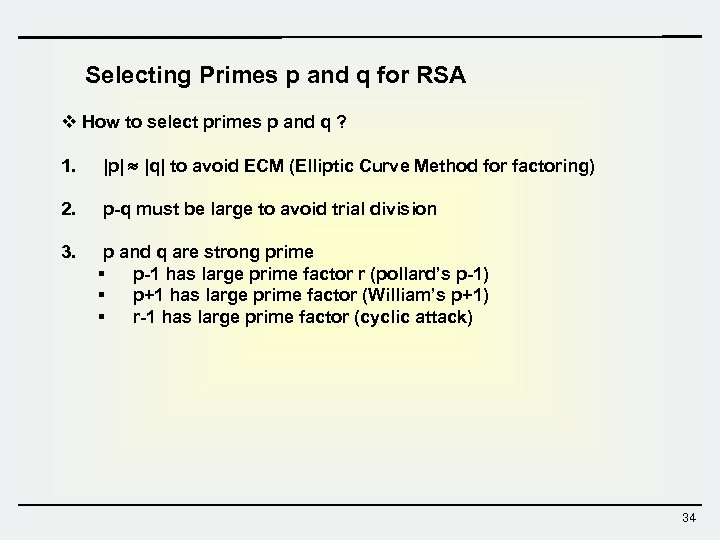Selecting Primes p and q for RSA v How to select primes p and q ? 1. |p| |q| to avoid ECM (Elliptic Curve Method for factoring) 2. p-q must be large to avoid trial division 3. p and q are strong prime § p-1 has large prime factor r (pollard’s p-1) § p+1 has large prime factor (William’s p+1) § r-1 has large prime factor (cyclic attack) 34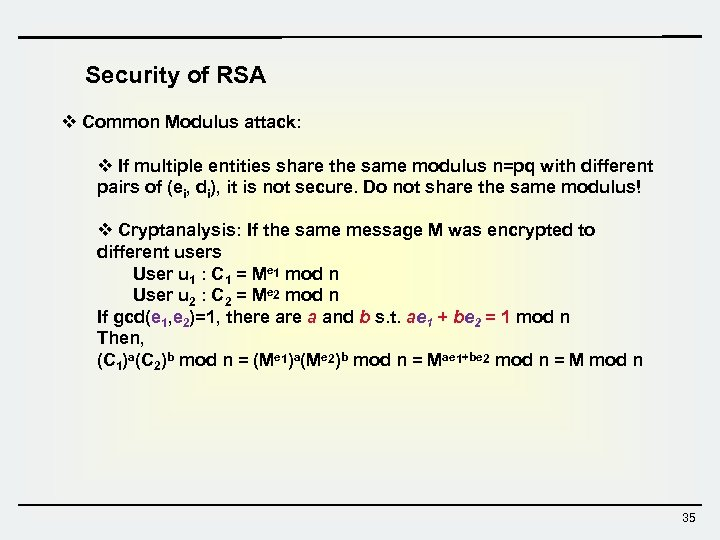Security of RSA v Common Modulus attack: v If multiple entities share the same modulus n=pq with different pairs of (ei, di), it is not secure. Do not share the same modulus! v Cryptanalysis: If the same message M was encrypted to different users User u 1 : C 1 = Me 1 mod n User u 2 : C 2 = Me 2 mod n If gcd(e 1, e 2)=1, there a and b s. t. ae 1 + be 2 = 1 mod n Then, (C 1)a(C 2)b mod n = (Me 1)a(Me 2)b mod n = Mae 1+be 2 mod n = M mod n 35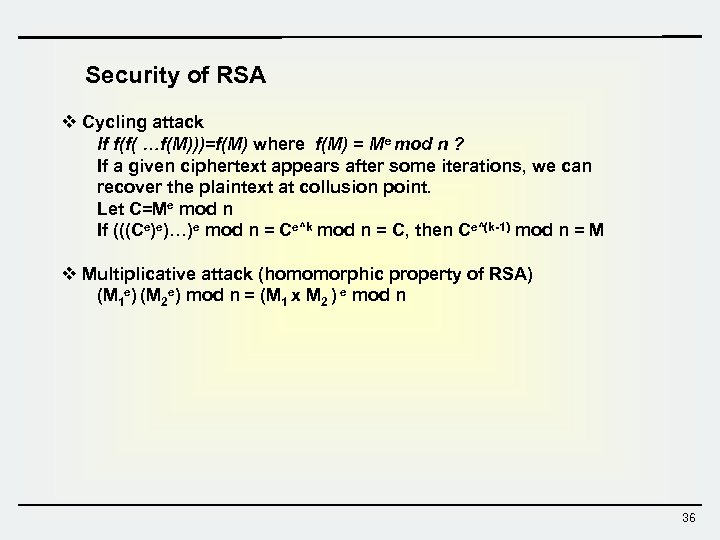Security of RSA v Cycling attack If f(f( …f(M)))=f(M) where f(M) = Me mod n ? If a given ciphertext appears after some iterations, we can recover the plaintext at collusion point. Let C=Me mod n If (((Ce)e)…)e mod n = Ce^k mod n = C, then Ce^(k-1) mod n = M v Multiplicative attack (homomorphic property of RSA) (M 1 e) (M 2 e) mod n = (M 1 x M 2 ) e mod n 36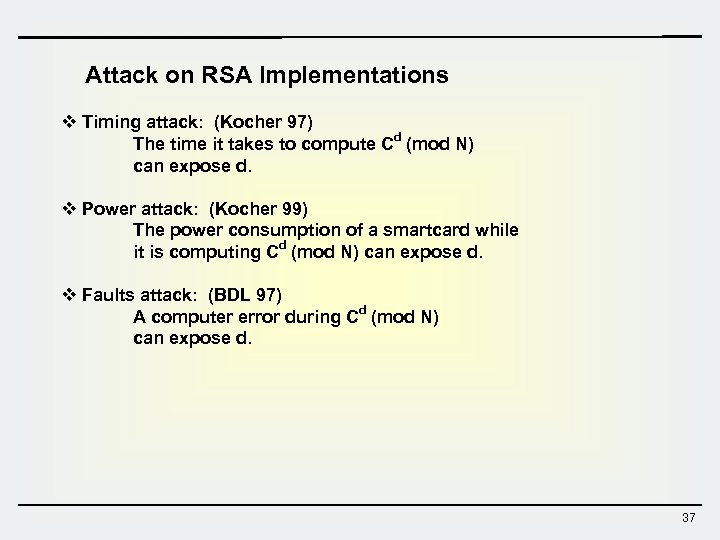Attack on RSA Implementations v Timing attack: (Kocher 97) The time it takes to compute Cd (mod N) can expose d. v Power attack: (Kocher 99) The power consumption of a smartcard while it is computing Cd (mod N) can expose d. v Faults attack: (BDL 97) A computer error during Cd (mod N) can expose d. 37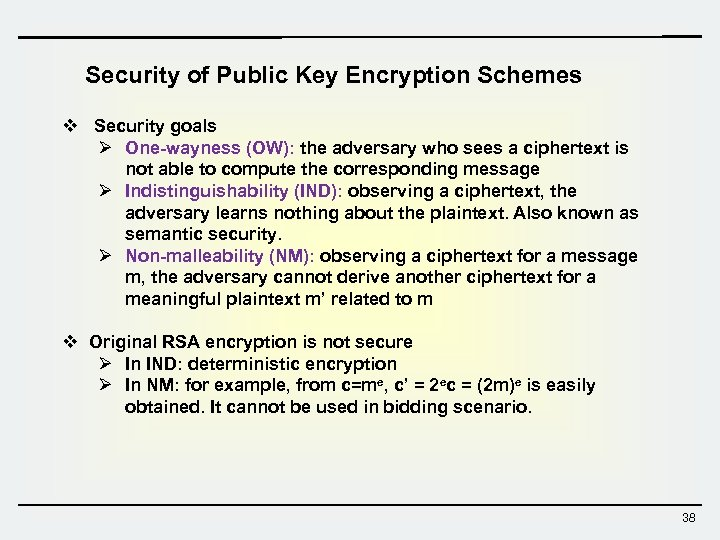Security of Public Key Encryption Schemes v Security goals Ø One-wayness (OW): the adversary who sees a ciphertext is not able to compute the corresponding message Ø Indistinguishability (IND): observing a ciphertext, the adversary learns nothing about the plaintext. Also known as semantic security. Ø Non-malleability (NM): observing a ciphertext for a message m, the adversary cannot derive another ciphertext for a meaningful plaintext m’ related to m v Original RSA encryption is not secure Ø In IND: deterministic encryption Ø In NM: for example, from c=me, c’ = 2 ec = (2 m)e is easily obtained. It cannot be used in bidding scenario. 38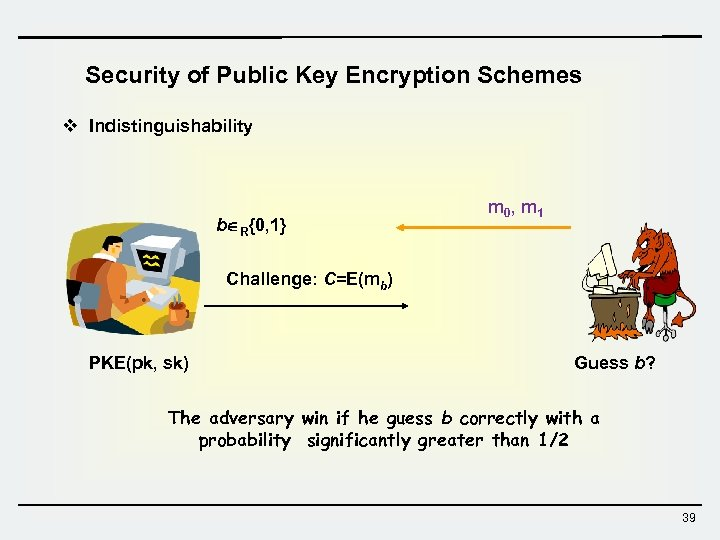Security of Public Key Encryption Schemes v Indistinguishability b R{0, 1} m 0 , m 1 Challenge: C=E(mb) PKE(pk, sk) Guess b? The adversary win if he guess b correctly with a probability significantly greater than 1/2 39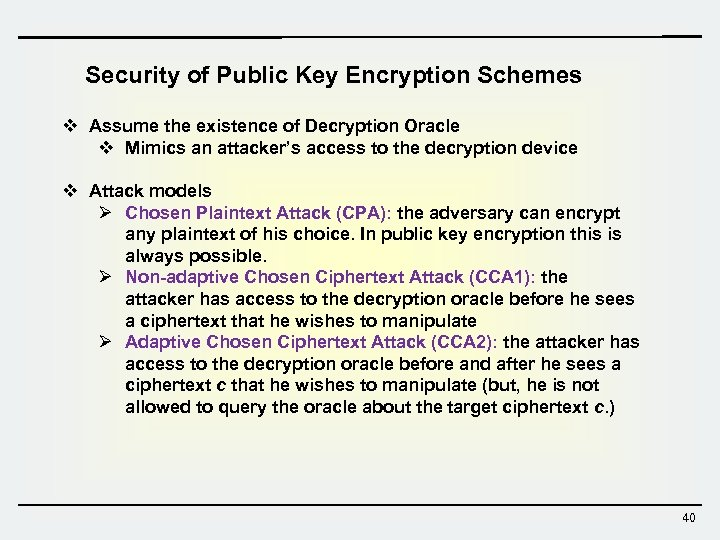Security of Public Key Encryption Schemes v Assume the existence of Decryption Oracle v Mimics an attacker’s access to the decryption device v Attack models Ø Chosen Plaintext Attack (CPA): the adversary can encrypt any plaintext of his choice. In public key encryption this is always possible. Ø Non-adaptive Chosen Ciphertext Attack (CCA 1): the attacker has access to the decryption oracle before he sees a ciphertext that he wishes to manipulate Ø Adaptive Chosen Ciphertext Attack (CCA 2): the attacker has access to the decryption oracle before and after he sees a ciphertext c that he wishes to manipulate (but, he is not allowed to query the oracle about the target ciphertext c. ) 40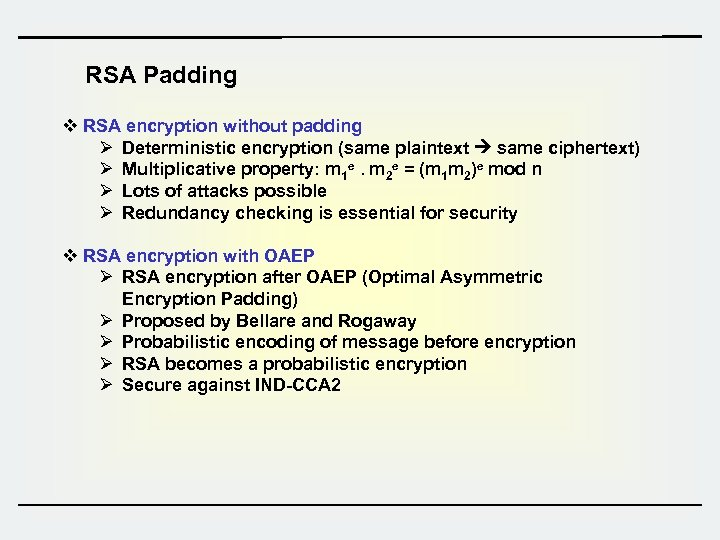RSA Padding v RSA encryption without padding Ø Deterministic encryption (same plaintext same ciphertext) Ø Multiplicative property: m 1 e. m 2 e = (m 1 m 2)e mod n Ø Lots of attacks possible Ø Redundancy checking is essential for security v RSA encryption with OAEP Ø RSA encryption after OAEP (Optimal Asymmetric Encryption Padding) Ø Proposed by Bellare and Rogaway Ø Probabilistic encoding of message before encryption Ø RSA becomes a probabilistic encryption Ø Secure against IND-CCA 2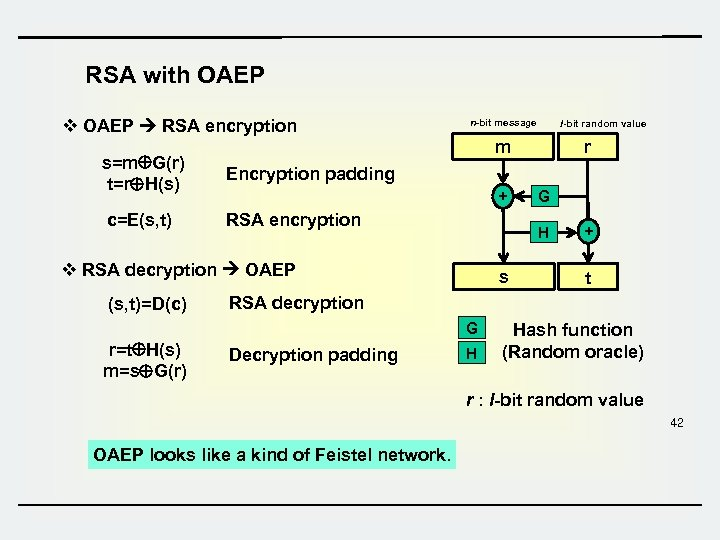RSA with OAEP v OAEP RSA encryption n-bit message l-bit random value m s=m G(r) t=r H(s) Encryption padding c=E(s, t) RSA encryption r + H v RSA decryption OAEP (s, t)=D(c) s + t RSA decryption G r=t H(s) m=s G(r) G Decryption padding H Hash function (Random oracle) r : l-bit random value 42 OAEP looks like a kind of Feistel network.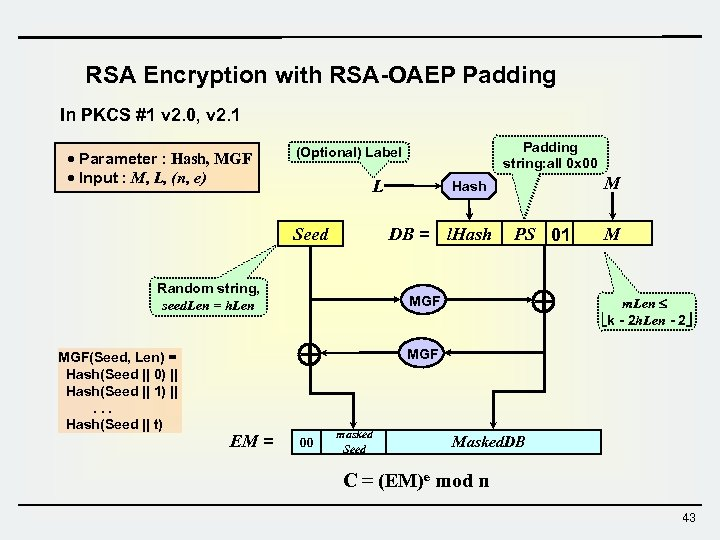RSA Encryption with RSA-OAEP Padding In PKCS #1 v 2. 0, v 2. 1 · Parameter : Hash, MGF · Input : M, L, (n, e) L Seed PS 01 MGF EM = M Hash DB = l. Hash Random string, seed. Len = h. Len MGF(Seed, Len) = Hash(Seed || 0) || Hash(Seed || 1) ||. . . Hash(Seed || t) Padding string: all 0 x 00 (Optional) Label 00 M m. Len k - 2 h. Len - 2 MGF masked Seed Masked. DB C = (EM)e mod n 43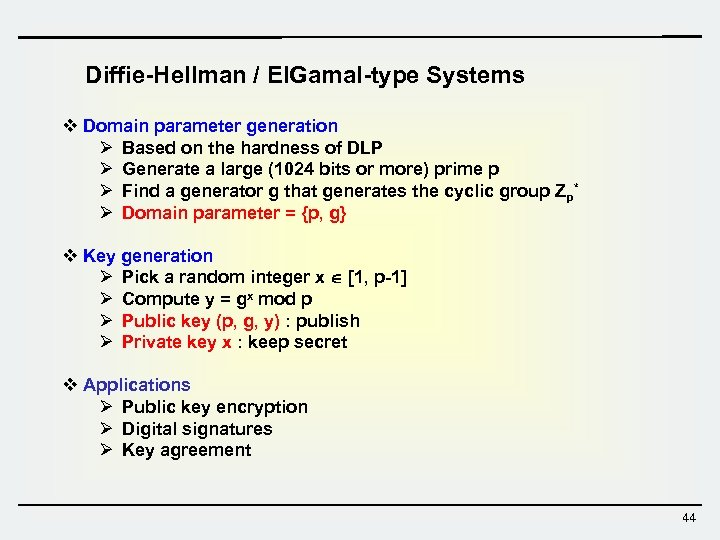Diffie-Hellman / El. Gamal-type Systems v Domain parameter generation Ø Based on the hardness of DLP Ø Generate a large (1024 bits or more) prime p Ø Find a generator g that generates the cyclic group Zp* Ø Domain parameter = {p, g} v Key generation Ø Pick a random integer x [1, p-1] Ø Compute y = gx mod p Ø Public key (p, g, y) : publish Ø Private key x : keep secret v Applications Ø Public key encryption Ø Digital signatures Ø Key agreement 44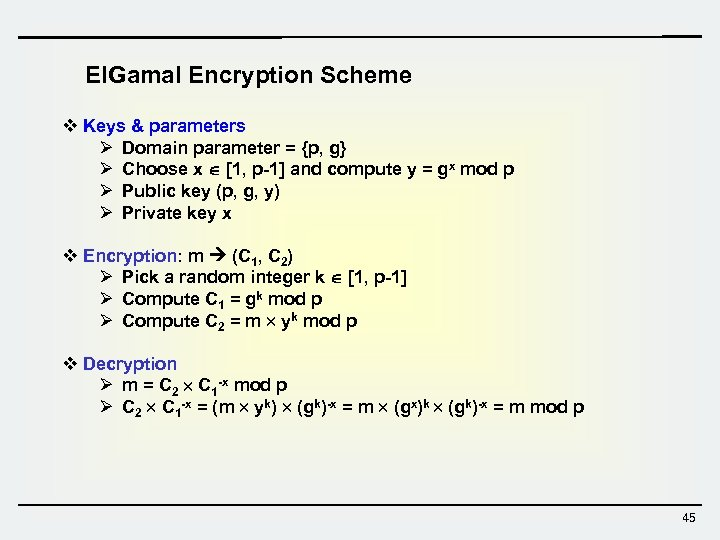El. Gamal Encryption Scheme v Keys & parameters Ø Domain parameter = {p, g} Ø Choose x [1, p-1] and compute y = gx mod p Ø Public key (p, g, y) Ø Private key x v Encryption: m (C 1, C 2) Ø Pick a random integer k [1, p-1] Ø Compute C 1 = gk mod p Ø Compute C 2 = m yk mod p v Decryption Ø m = C 2 C 1 -x mod p Ø C 2 C 1 -x = (m yk) (gk)-x = m (gx)k (gk)-x = m mod p 45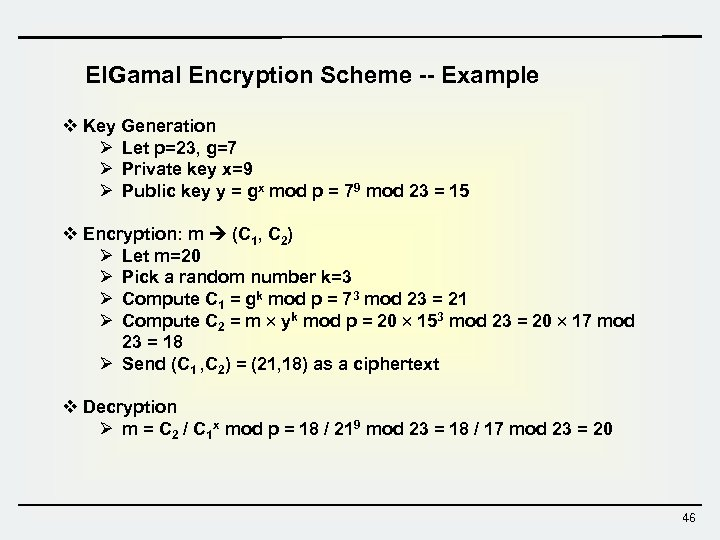El. Gamal Encryption Scheme -- Example v Key Generation Ø Let p=23, g=7 Ø Private key x=9 Ø Public key y = gx mod p = 79 mod 23 = 15 v Encryption: m (C 1, C 2) Ø Let m=20 Ø Pick a random number k=3 Ø Compute C 1 = gk mod p = 73 mod 23 = 21 Ø Compute C 2 = m yk mod p = 20 153 mod 23 = 20 17 mod 23 = 18 Ø Send (C 1 , C 2) = (21, 18) as a ciphertext v Decryption Ø m = C 2 / C 1 x mod p = 18 / 219 mod 23 = 18 / 17 mod 23 = 20 46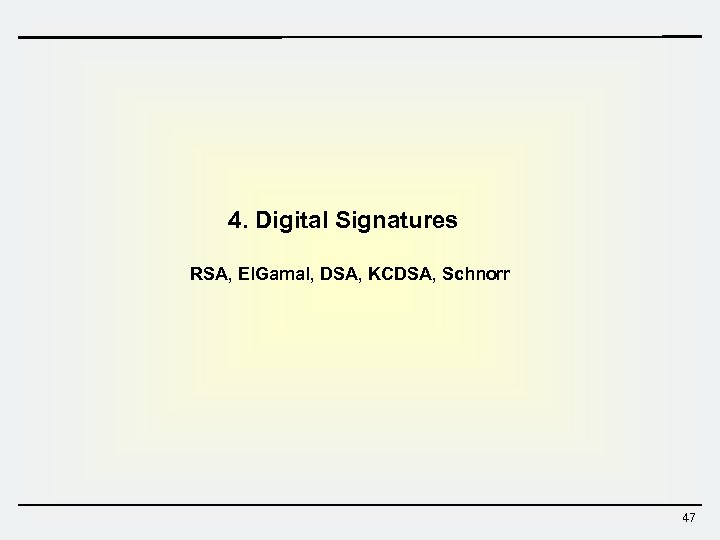4. Digital Signatures RSA, El. Gamal, DSA, KCDSA, Schnorr 47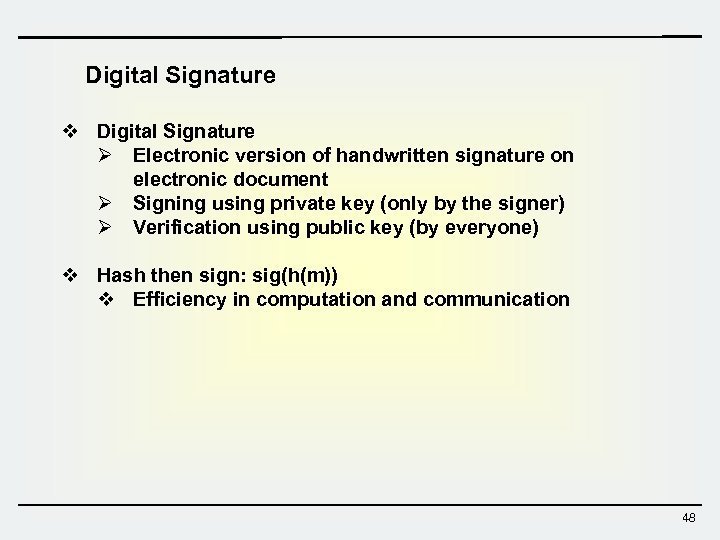Digital Signature v Digital Signature Ø Electronic version of handwritten signature on electronic document Ø Signing using private key (only by the signer) Ø Verification using public key (by everyone) v Hash then sign: sig(h(m)) v Efficiency in computation and communication 48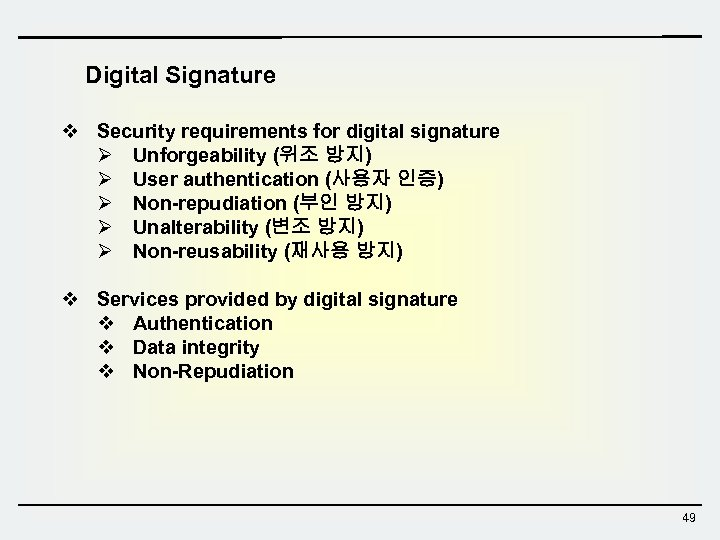Digital Signature v Security requirements for digital signature Ø Unforgeability (위조 방지) Ø User authentication (사용자 인증) Ø Non-repudiation (부인 방지) Ø Unalterability (변조 방지) Ø Non-reusability (재사용 방지) v Services provided by digital signature v Authentication v Data integrity v Non-Repudiation 49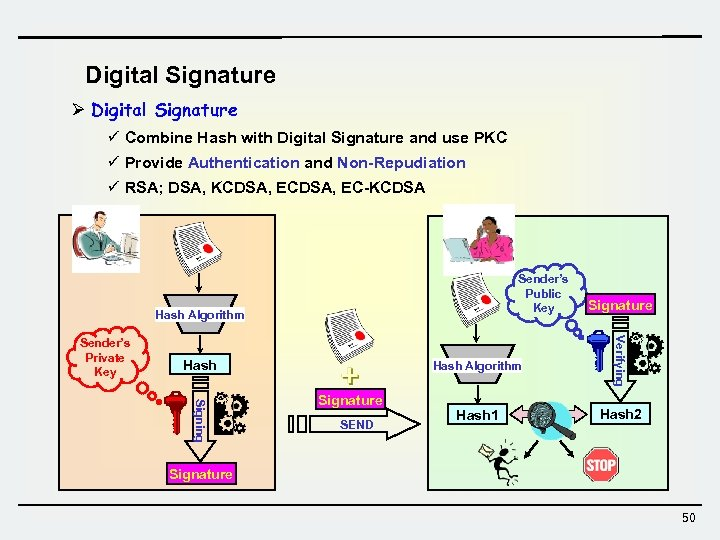Digital Signature Ø Digital Signature ü Combine Hash with Digital Signature and use PKC ü Provide Authentication and Non-Repudiation ü RSA; DSA, KCDSA, EC-KCDSA Sender’s Public Key Hash Algorithm Hash Signing Signature SEND Verifying Sender’s Private Key Signature Hash 1 Hash 2 Signature 50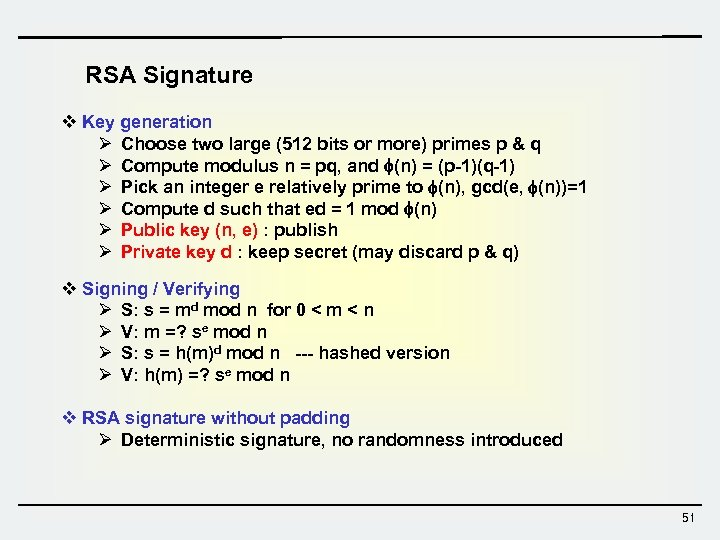RSA Signature v Key generation Ø Choose two large (512 bits or more) primes p & q Ø Compute modulus n = pq, and (n) = (p-1)(q-1) Ø Pick an integer e relatively prime to (n), gcd(e, (n))=1 Ø Compute d such that ed = 1 mod (n) Ø Public key (n, e) : publish Ø Private key d : keep secret (may discard p & q) v Signing / Verifying Ø S: s = md mod n for 0 < m < n Ø V: m =? se mod n Ø S: s = h(m)d mod n --- hashed version Ø V: h(m) =? se mod n v RSA signature without padding Ø Deterministic signature, no randomness introduced 51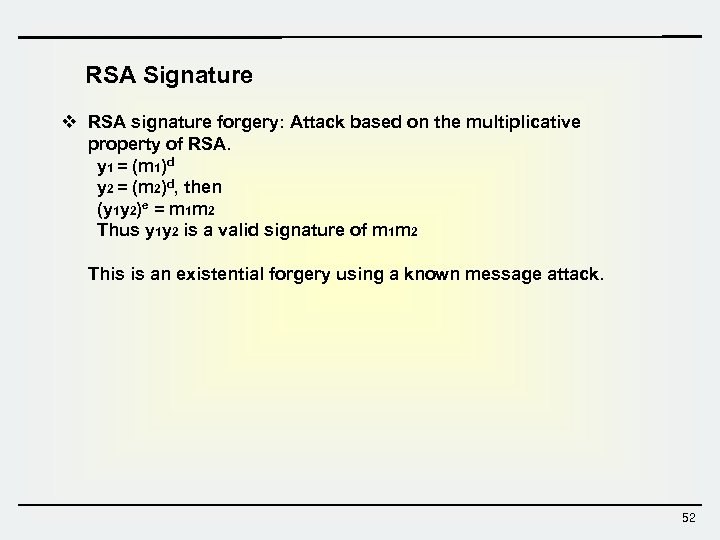RSA Signature v RSA signature forgery: Attack based on the multiplicative property of RSA. y 1 = (m 1)d y 2 = (m 2)d, then (y 1 y 2)e = m 1 m 2 Thus y 1 y 2 is a valid signature of m 1 m 2 This is an existential forgery using a known message attack. 52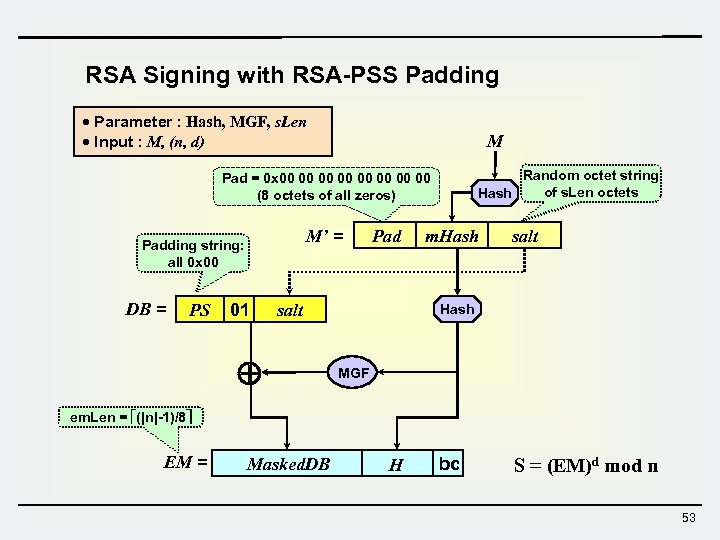RSA Signing with RSA-PSS Padding · Parameter : Hash, MGF, s. Len · Input : M, (n, d) M Random octet string of s. Len octets Hash Pad = 0 x 00 00 (8 octets of all zeros) M’ = Padding string: all 0 x 00 DB = PS 01 Pad salt m. Hash salt Hash MGF em. Len = (|n|-1)/8 EM = Masked. DB H bc S = (EM)d mod n 53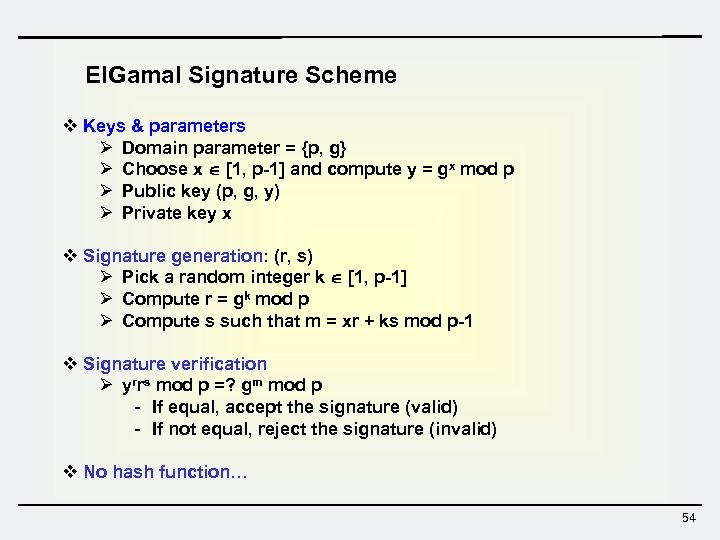El. Gamal Signature Scheme v Keys & parameters Ø Domain parameter = {p, g} Ø Choose x [1, p-1] and compute y = gx mod p Ø Public key (p, g, y) Ø Private key x v Signature generation: (r, s) Ø Pick a random integer k [1, p-1] Ø Compute r = gk mod p Ø Compute s such that m = xr + ks mod p-1 v Signature verification Ø yrrs mod p =? gm mod p - If equal, accept the signature (valid) - If not equal, reject the signature (invalid) v No hash function… 54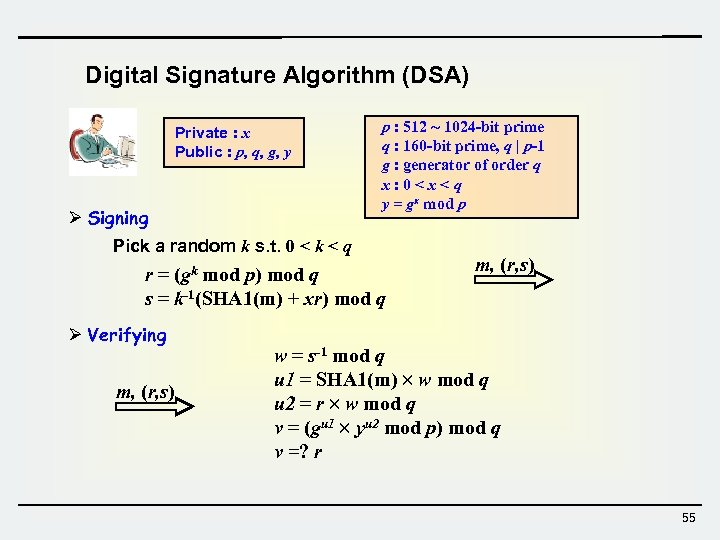Digital Signature Algorithm (DSA) Private : x Public : p, q, g, y Ø Signing p : 512 ~ 1024 -bit prime q : 160 -bit prime, q | p-1 g : generator of order q x: 0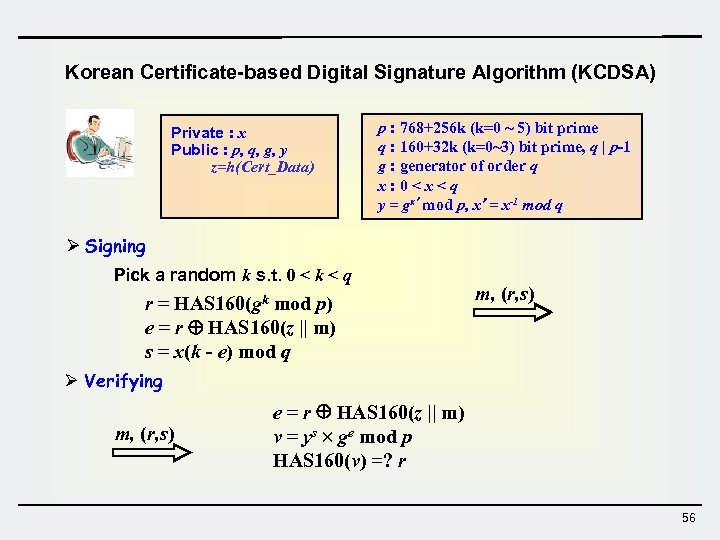Korean Certificate-based Digital Signature Algorithm (KCDSA) Private : x Public : p, q, g, y z=h(Cert_Data) p : 768+256 k (k=0 ~ 5) bit prime q : 160+32 k (k=0~3) bit prime, q | p-1 g : generator of order q x: 0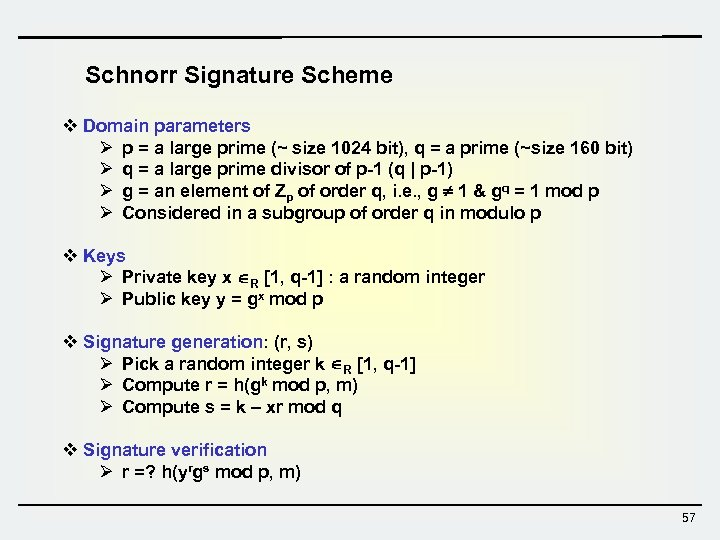Schnorr Signature Scheme v Domain parameters Ø p = a large prime (~ size 1024 bit), q = a prime (~size 160 bit) Ø q = a large prime divisor of p-1 (q | p-1) Ø g = an element of Zp of order q, i. e. , g 1 & gq = 1 mod p Ø Considered in a subgroup of order q in modulo p v Keys Ø Private key x R [1, q-1] : a random integer Ø Public key y = gx mod p v Signature generation: (r, s) Ø Pick a random integer k R [1, q-1] Ø Compute r = h(gk mod p, m) Ø Compute s = k – xr mod q v Signature verification Ø r =? h(yrgs mod p, m) 57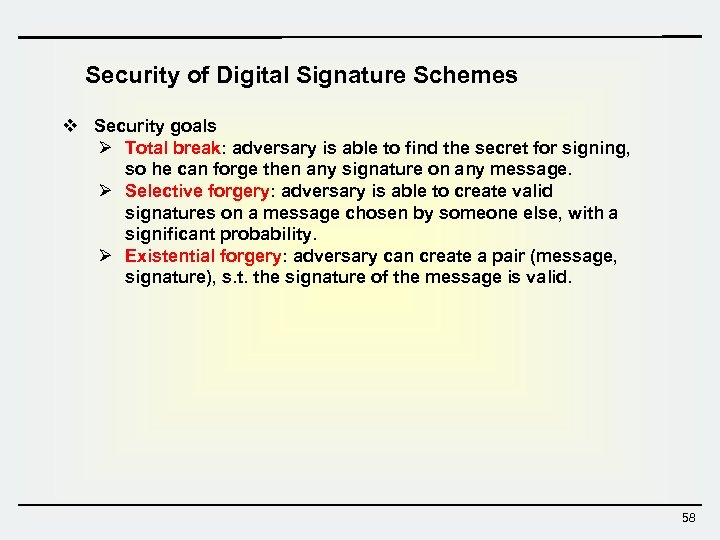Security of Digital Signature Schemes v Security goals Ø Total break: adversary is able to find the secret for signing, so he can forge then any signature on any message. Ø Selective forgery: adversary is able to create valid signatures on a message chosen by someone else, with a significant probability. Ø Existential forgery: adversary can create a pair (message, signature), s. t. the signature of the message is valid. 58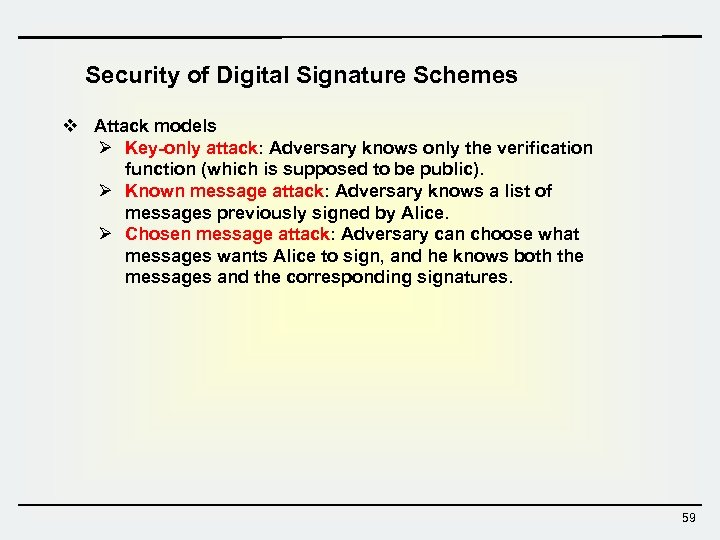Security of Digital Signature Schemes v Attack models Ø Key-only attack: Adversary knows only the verification function (which is supposed to be public). Ø Known message attack: Adversary knows a list of messages previously signed by Alice. Ø Chosen message attack: Adversary can choose what messages wants Alice to sign, and he knows both the messages and the corresponding signatures. 59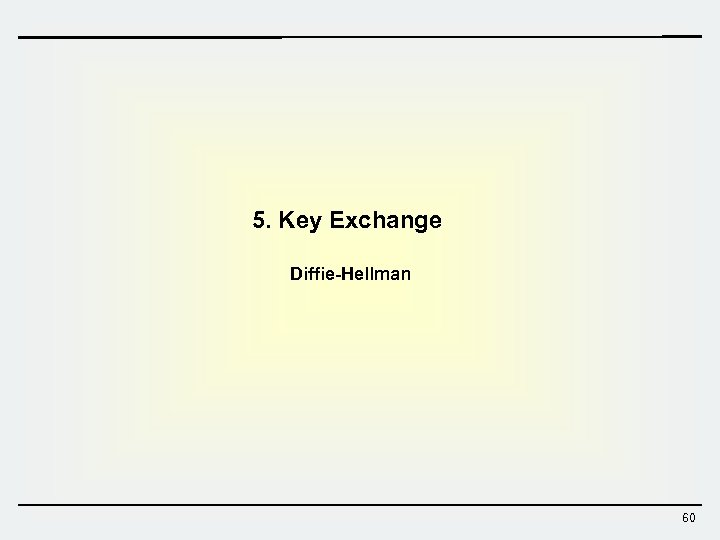5. Key Exchange Diffie-Hellman 60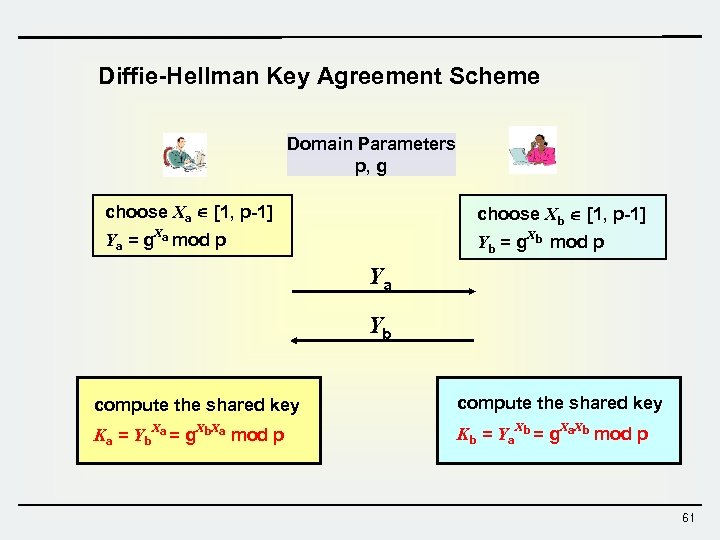Diffie-Hellman Key Agreement Scheme Domain Parameters p, g choose Xa [1, p-1] choose Xb [1, p-1] Ya = g. Xa mod p Yb = g. Xb mod p Ya Yb compute the shared key Ka = Yb. Xa = g. Xb. Xa mod p Kb = Ya. Xb = g. Xa. Xb mod p 61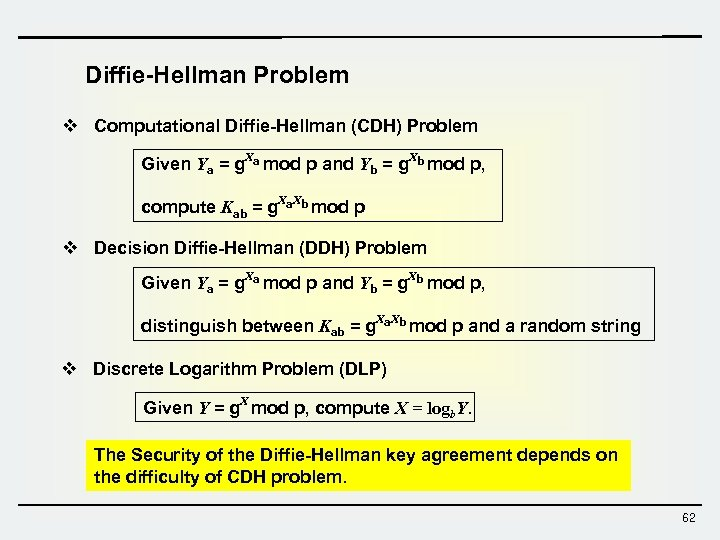Diffie-Hellman Problem v Computational Diffie-Hellman (CDH) Problem Given Ya = g. Xa mod p and Yb = g. Xb mod p, compute Kab = g. Xa. Xb mod p v Decision Diffie-Hellman (DDH) Problem Given Ya = g. Xa mod p and Yb = g. Xb mod p, distinguish between Kab = g. Xa. Xb mod p and a random string v Discrete Logarithm Problem (DLP) Given Y = g. X mod p, compute X = logb. Y. The Security of the Diffie-Hellman key agreement depends on the difficulty of CDH problem. 62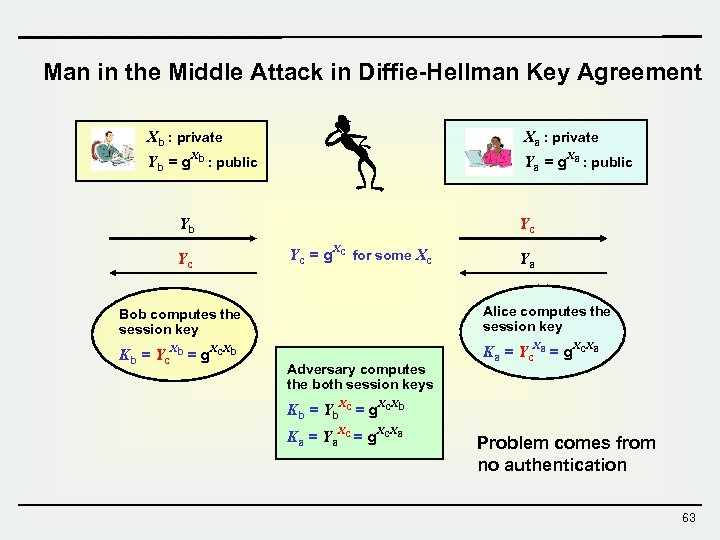Man in the Middle Attack in Diffie-Hellman Key Agreement Xb : private Xa : private Yb = g. Xb : public Ya = g. Xa : public Yb Yc Yc Yc = g. Xc for some Xc Ya Bob computes the session key Alice computes the session key Kb = Yc. Xb = g. Xc. Xb Ka = Yc. Xa = g. Xc. Xa Adversary computes the both session keys Kb = Yb. Xc = g. Xc. Xb Ka = Ya. Xc = g. Xc. Xa Problem comes from no authentication 63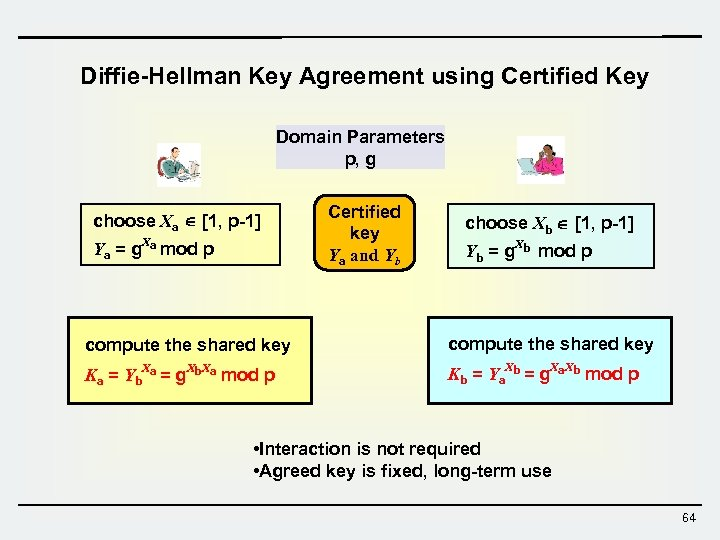Diffie-Hellman Key Agreement using Certified Key Domain Parameters p, g choose Xa [1, p-1] Ya = g. Xa mod p Certified key Ya and Yb choose Xb [1, p-1] Yb = g. Xb mod p compute the shared key Ka = Yb. Xa = g. Xb. Xa mod p Kb = Ya. Xb = g. Xa. Xb mod p • Interaction is not required • Agreed key is fixed, long-term use 64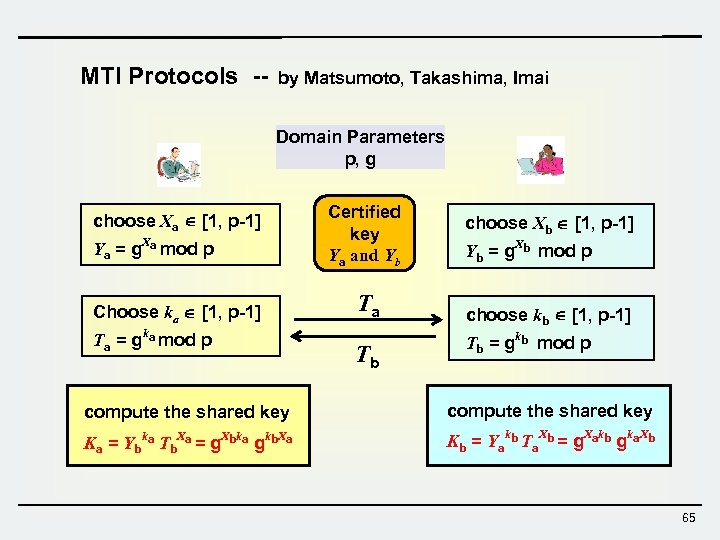MTI Protocols -- by Matsumoto, Takashima, Imai Domain Parameters p, g choose Xa [1, p-1] Ya = g. Xa mod p Choose ka [1, p-1] Ta = gka mod p Certified key Ya and Yb Ta Tb choose Xb [1, p-1] Yb = g. Xb mod p choose kb [1, p-1] Tb = gkb mod p compute the shared key Ka = Ybka Tb. Xa = g. Xbka gkb. Xa Kb = Yakb Ta. Xb = g. Xakb gka. Xb 65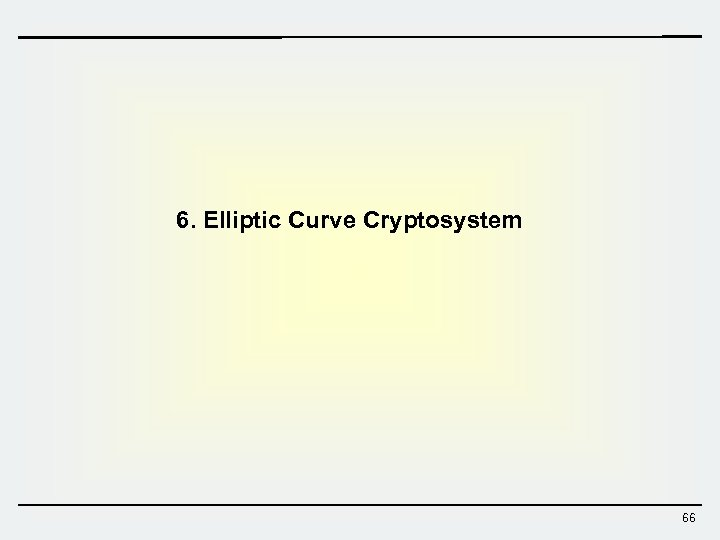6. Elliptic Curve Cryptosystem 66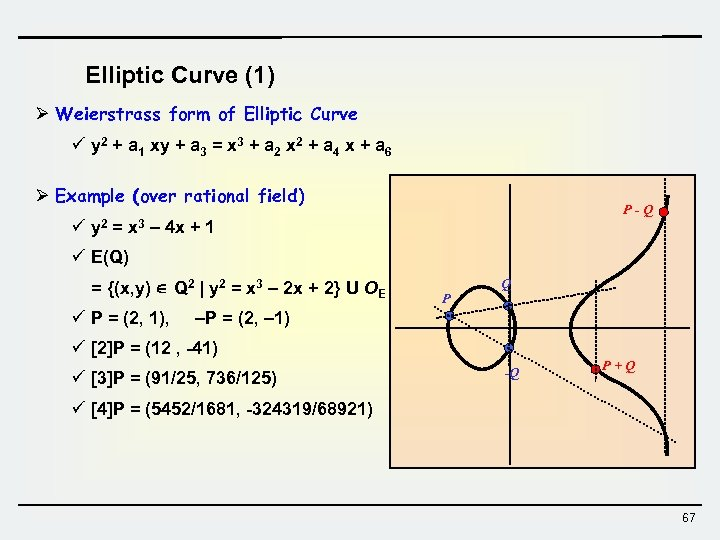Elliptic Curve (1) Ø Weierstrass form of Elliptic Curve ü y 2 + a 1 xy + a 3 = x 3 + a 2 x 2 + a 4 x + a 6 Ø Example (over rational field) ü y 2 = x 3 P-Q – 4 x + 1 ü E(Q) = {(x, y) Q 2 | y 2 = x 3 – 2 x + 2} U OE ü P = (2, 1), P Q –P = (2, – 1) ü P = (12 , -41) ü P = (91/25, 736/125) -Q P+Q ü P = (5452/1681, -324319/68921) 67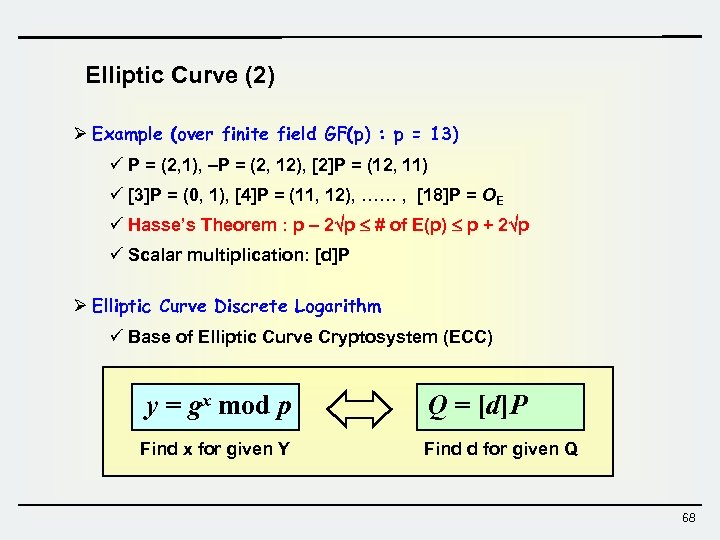Elliptic Curve (2) Ø Example (over finite field GF(p) : p = 13) ü P = (2, 1), –P = (2, 12), P = (12, 11) ü P = (0, 1), P = (11, 12), …… , P = OE ü Hasse’s Theorem : p – 2 p # of E(p) p + 2 p ü Scalar multiplication: [d]P Ø Elliptic Curve Discrete Logarithm ü Base of Elliptic Curve Cryptosystem (ECC) y = gx mod p Find x for given Y Q = [d]P Find d for given Q 68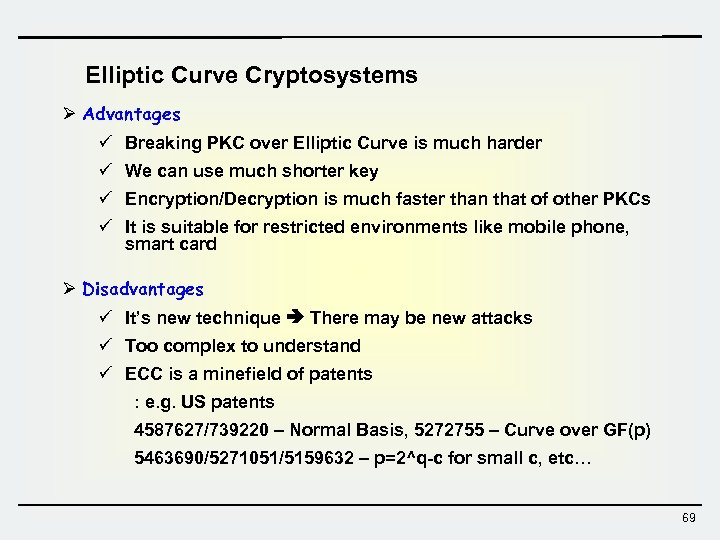Elliptic Curve Cryptosystems Ø Advantages ü Breaking PKC over Elliptic Curve is much harder ü We can use much shorter key ü Encryption/Decryption is much faster than that of other PKCs ü It is suitable for restricted environments like mobile phone, smart card Ø Disadvantages ü It’s new technique There may be new attacks ü Too complex to understand ü ECC is a minefield of patents : e. g. US patents 4587627/739220 – Normal Basis, 5272755 – Curve over GF(p) 5463690/5271051/5159632 – p=2^q-c for small c, etc… 69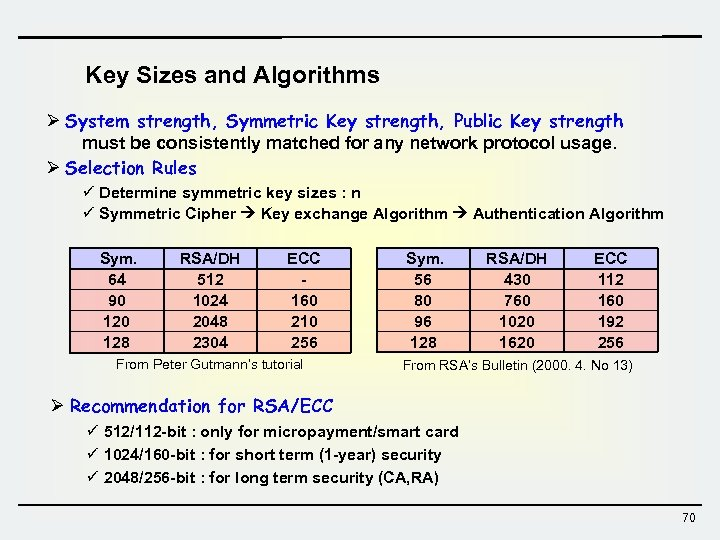Key Sizes and Algorithms Ø System strength, Symmetric Key strength, Public Key strength must be consistently matched for any network protocol usage. Ø Selection Rules ü Determine symmetric key sizes : n ü Symmetric Cipher Key exchange Algorithm Authentication Algorithm Sym. 64 90 128 RSA/DH 512 1024 2048 2304 ECC 160 210 256 From Peter Gutmann’s tutorial Sym. 56 80 96 128 RSA/DH 430 760 1020 1620 ECC 112 160 192 256 From RSA’s Bulletin (2000. 4. No 13) Ø Recommendation for RSA/ECC ü 512/112 -bit : only for micropayment/smart card ü 1024/160 -bit : for short term (1 -year) security ü 2048/256 -bit : for long term security (CA, RA) 70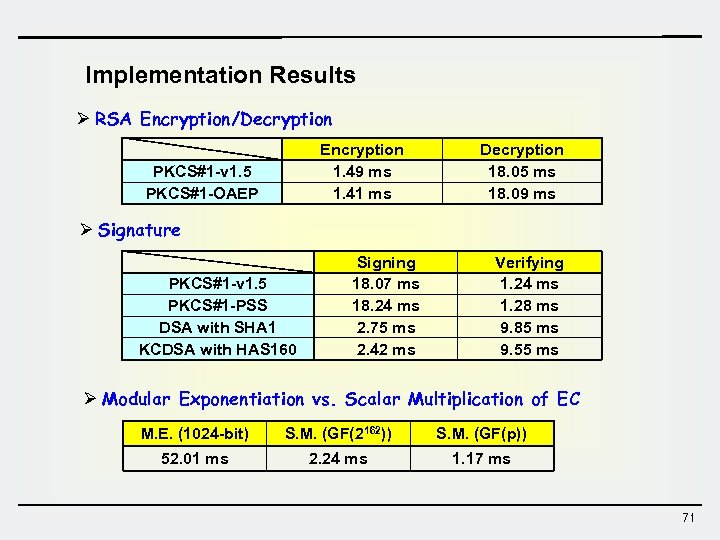Implementation Results Ø RSA Encryption/Decryption Encryption 1. 49 ms 1. 41 ms PKCS#1 -v 1. 5 PKCS#1 -OAEP Decryption 18. 05 ms 18. 09 ms Ø Signature PKCS#1 -v 1. 5 PKCS#1 -PSS DSA with SHA 1 KCDSA with HAS 160 Signing 18. 07 ms 18. 24 ms 2. 75 ms 2. 42 ms Verifying 1. 24 ms 1. 28 ms 9. 85 ms 9. 55 ms Ø Modular Exponentiation vs. Scalar Multiplication of EC M. E. (1024 -bit) S. M. (GF(2162)) S. M. (GF(p)) 52. 01 ms 2. 24 ms 1. 17 ms 71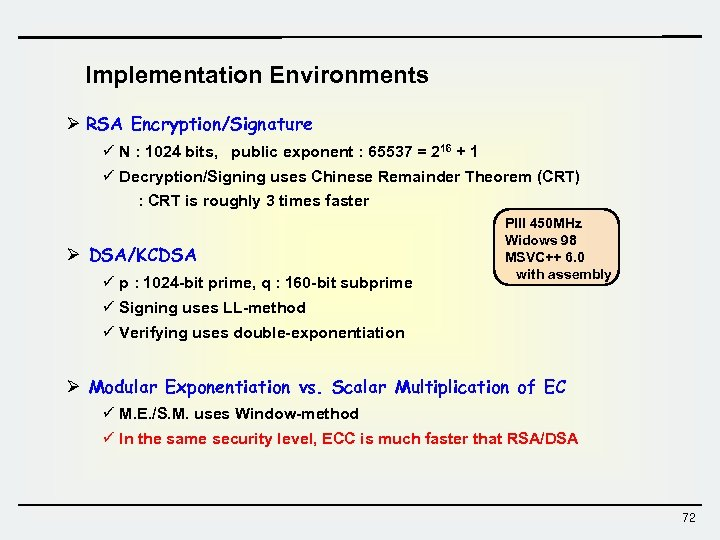Implementation Environments Ø RSA Encryption/Signature ü N : 1024 bits, public exponent : 65537 = 216 + 1 ü Decryption/Signing uses Chinese Remainder Theorem (CRT) : CRT is roughly 3 times faster Ø DSA/KCDSA ü p : 1024 -bit prime, q : 160 -bit subprime PIII 450 MHz Widows 98 MSVC++ 6. 0 with assembly ü Signing uses LL-method ü Verifying uses double-exponentiation Ø Modular Exponentiation vs. Scalar Multiplication of EC ü M. E. /S. M. uses Window-method ü In the same security level, ECC is much faster that RSA/DSA 72